# Generalized Matrix Decomposition

##### Posted on Jan 17, 2020 (Update: Feb 15, 2020)

This post is based on the talk given by Dr. Yue Wang at the Department of Statistics and Data Science, Southern University of Science and Technology on Jan. 04, 2020.

## Motivation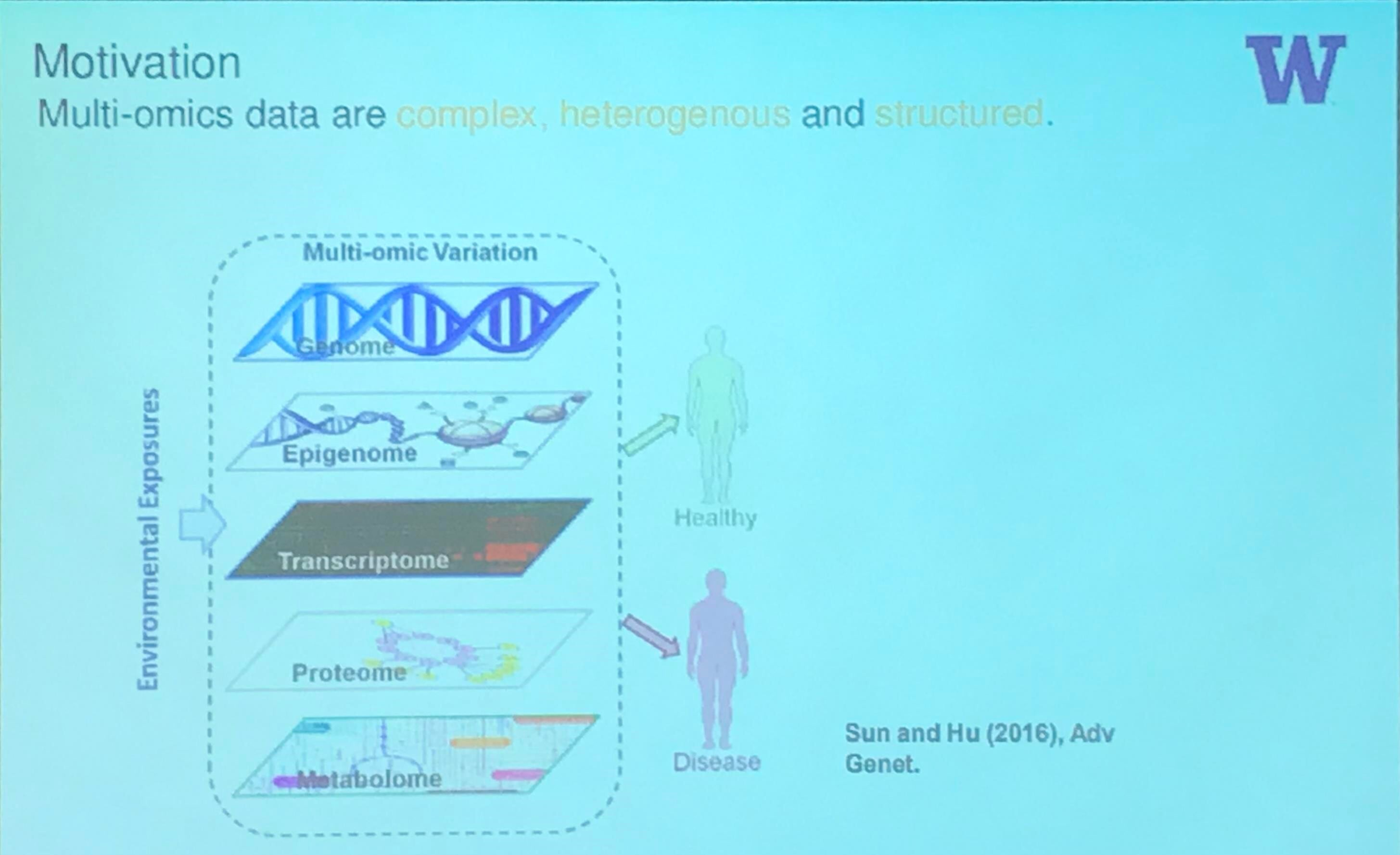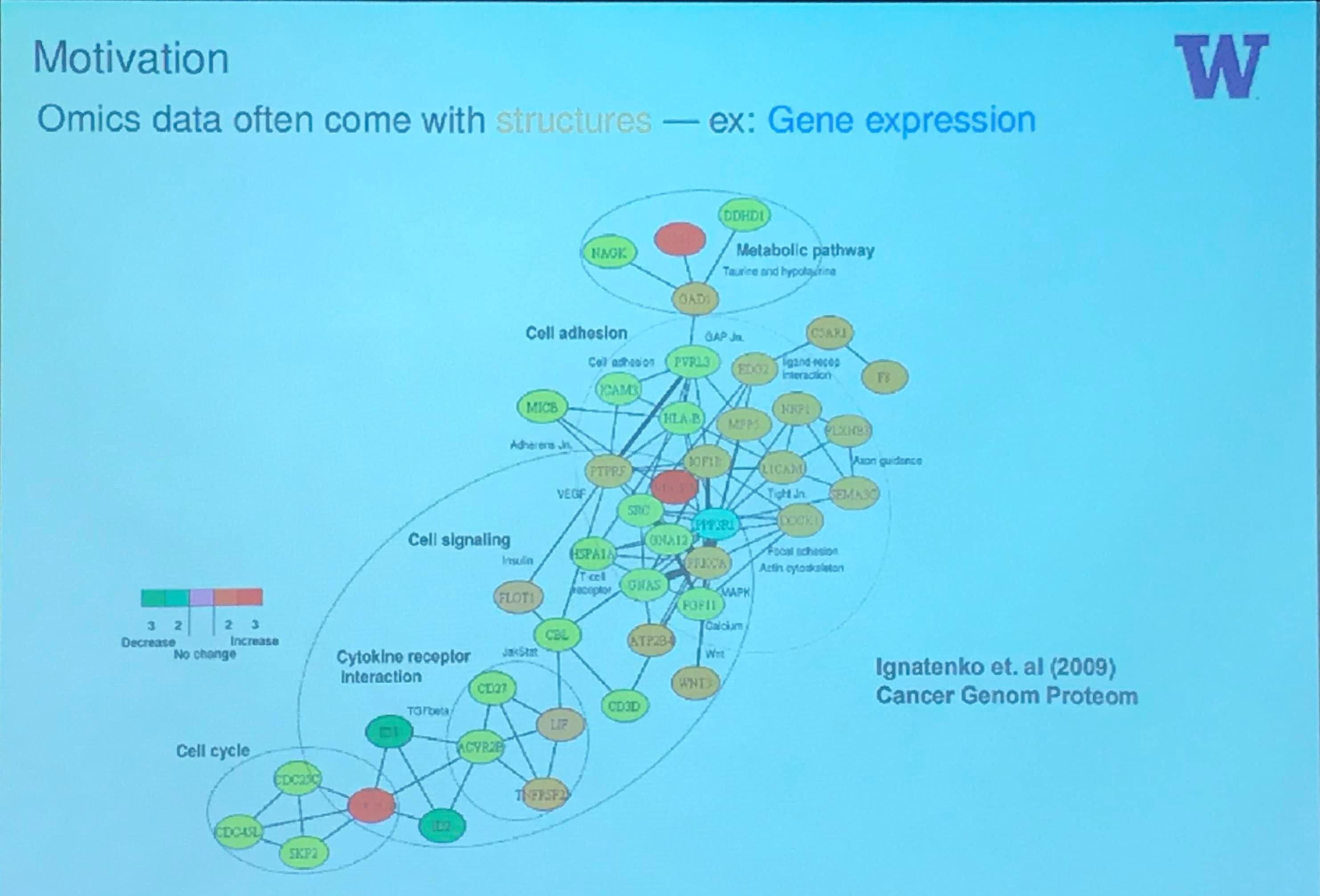## Microbiome Data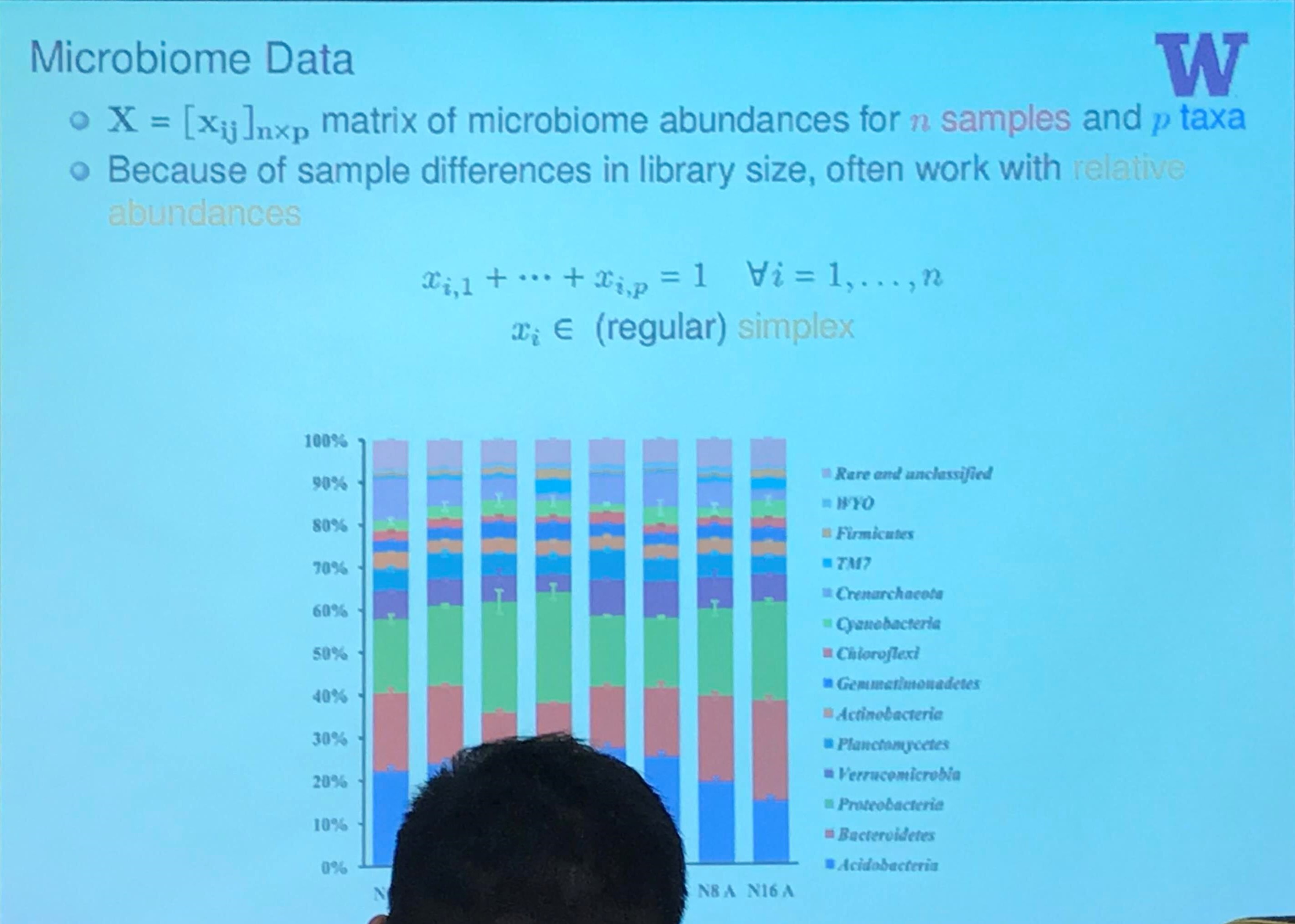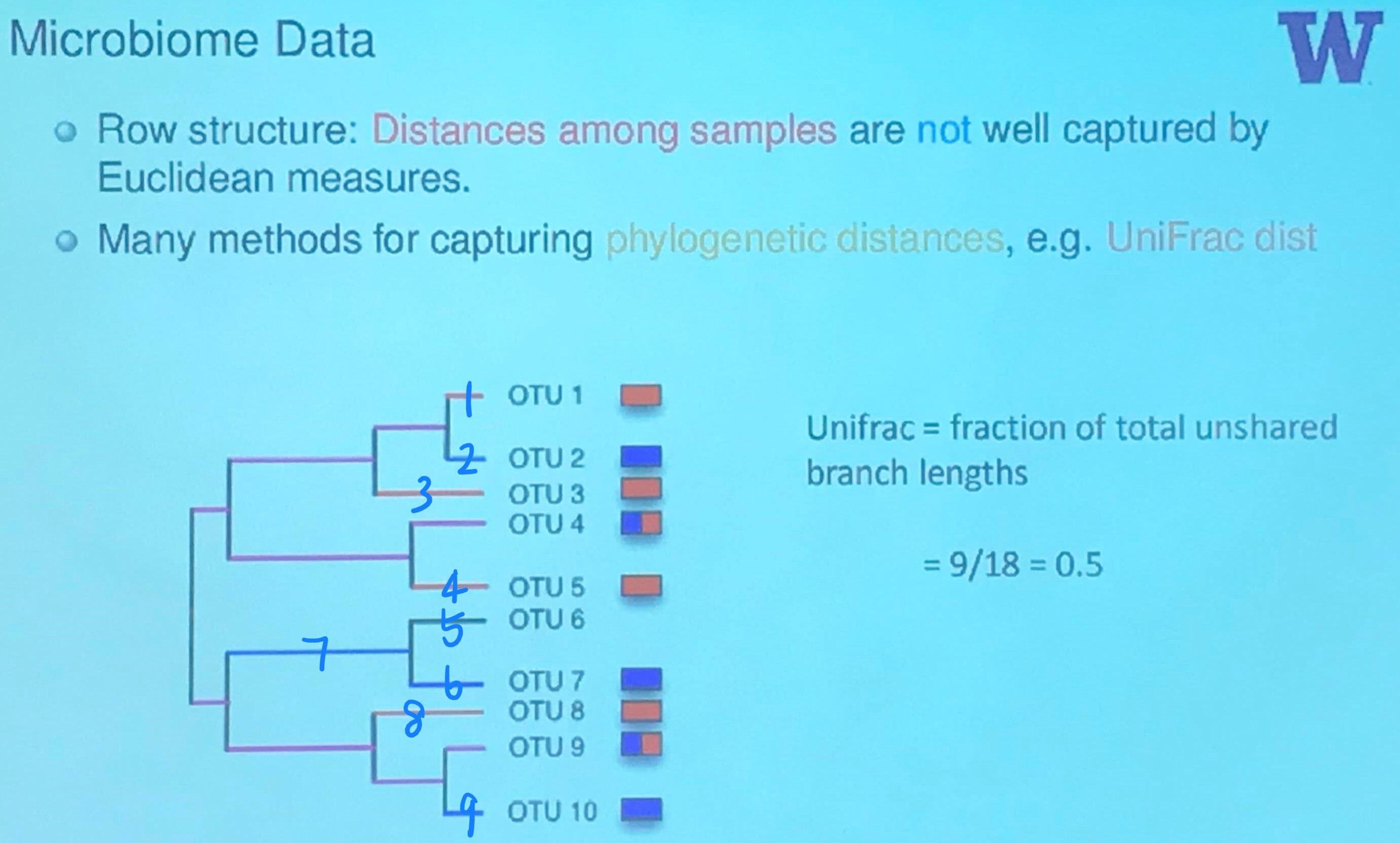Here is a great tutorial with python code to calculate $\beta$ diversity, including the UniFrac, and Bray-Curtis.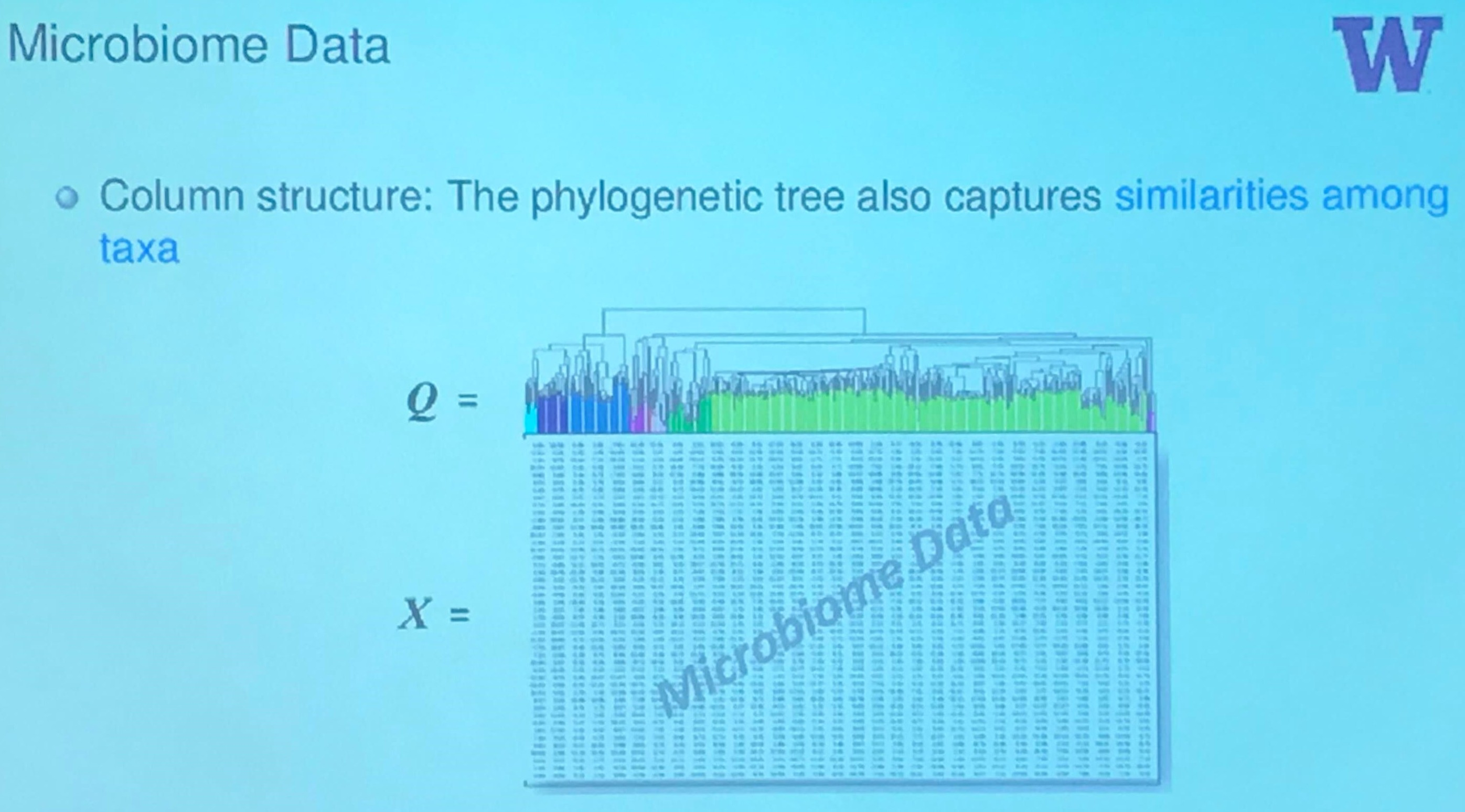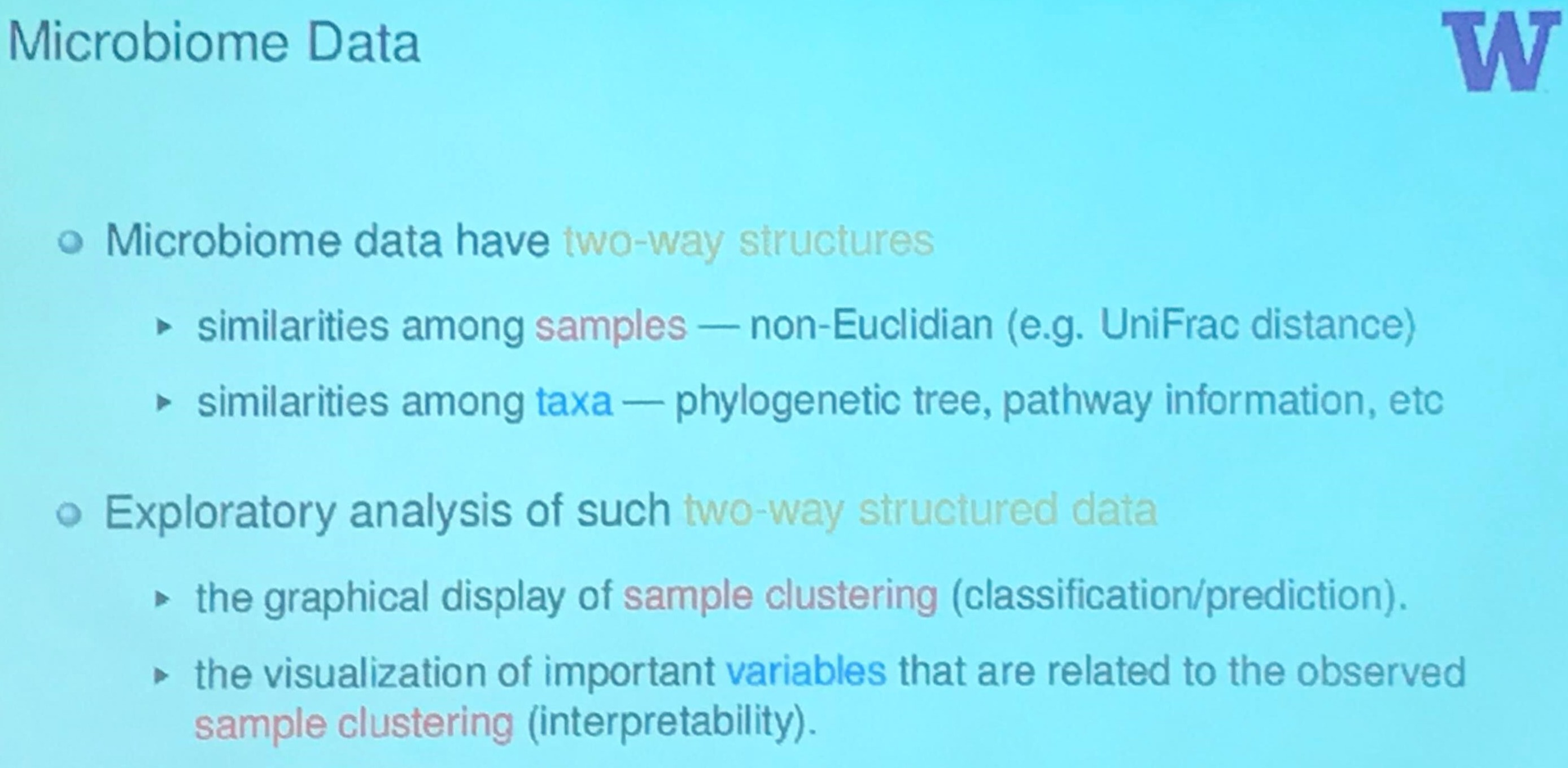## Exploratory Analysis: Sample Clustering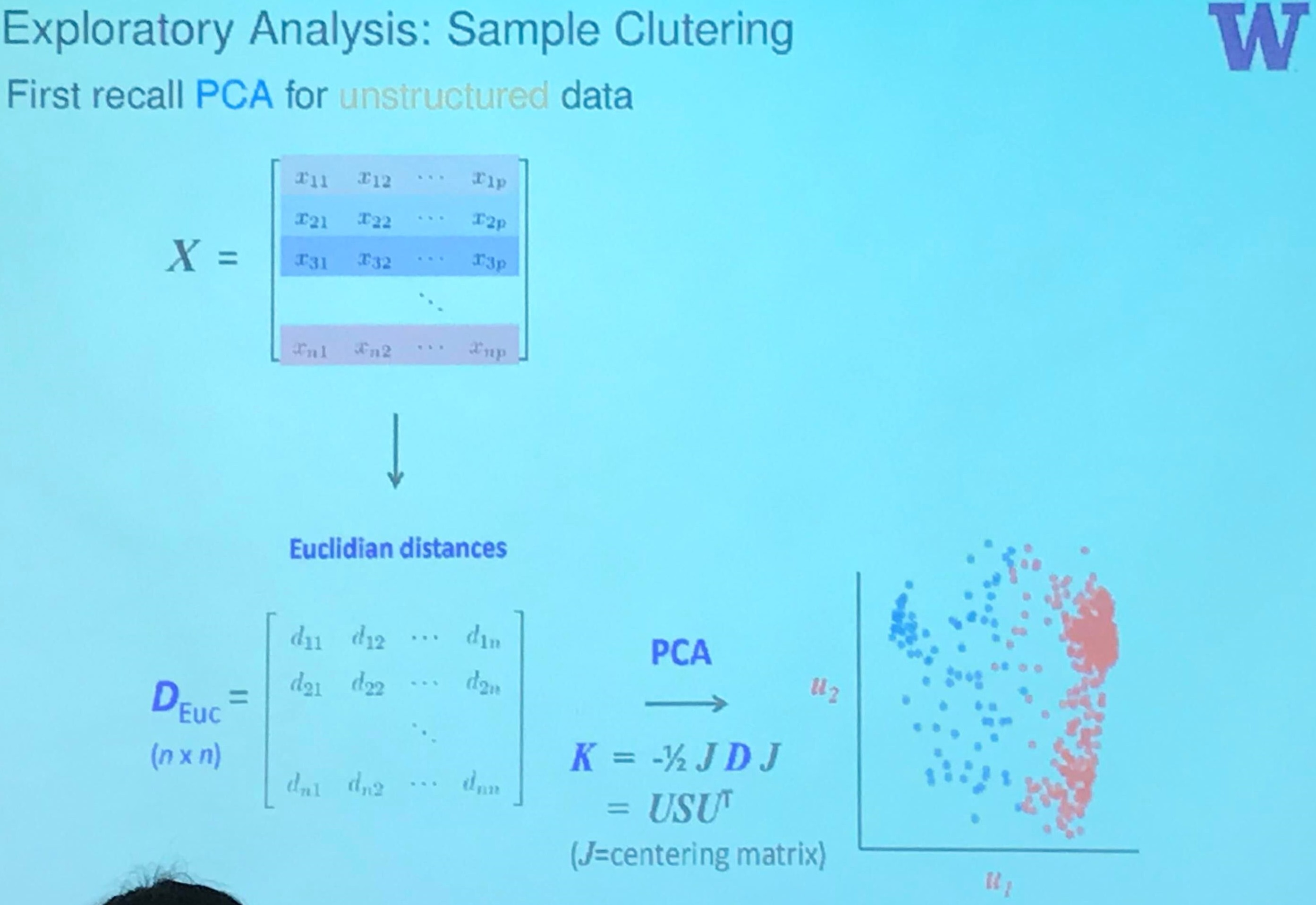Actually, it should be treated as classical scaling, an approach of multidimensional scaling (MDS), but the classical scaling is equivalent to the principal analysis if the similarity is defined as the centered inner-products.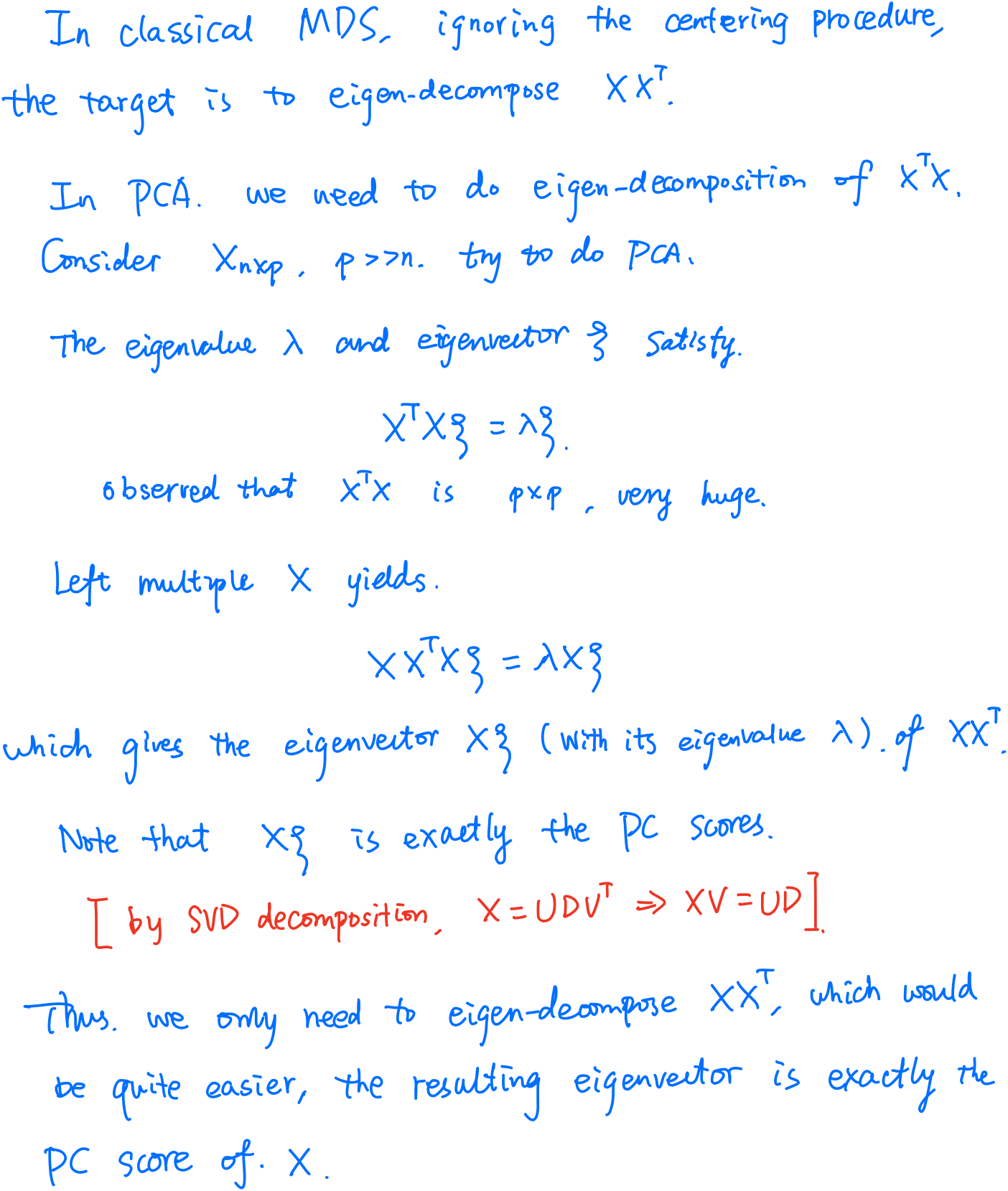But here is still some differences. In the slide, it is the distance, the square root of the inner-product. And note that if $X=USV^T$, then $X^TX = VD^2V^T$ and $XX^T=UD^2U^T$.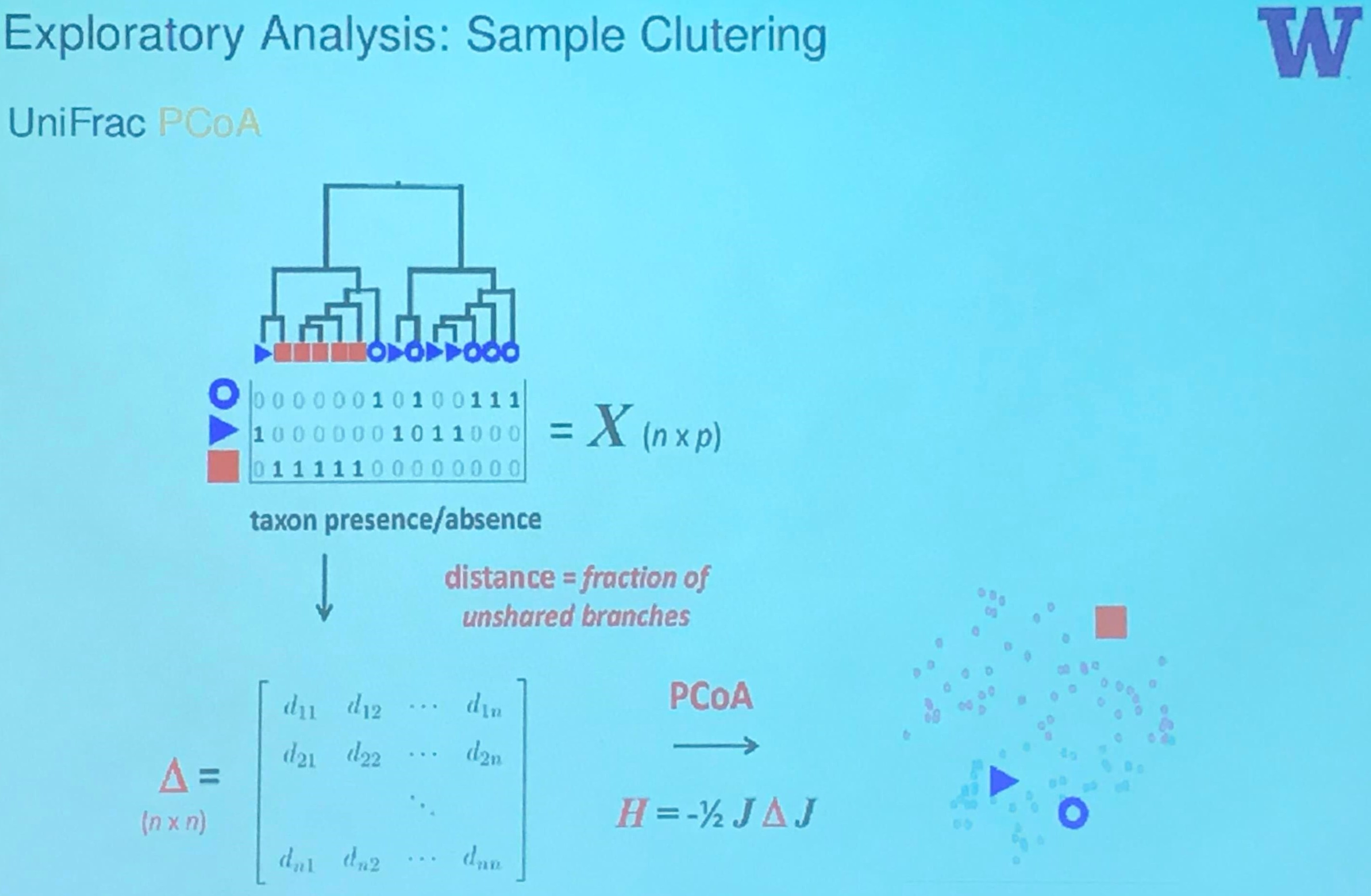The wikipedia page of MDS says that MDS is also known as Principal Coordinate Analysis.

## Exploratory Analysis: Important Variables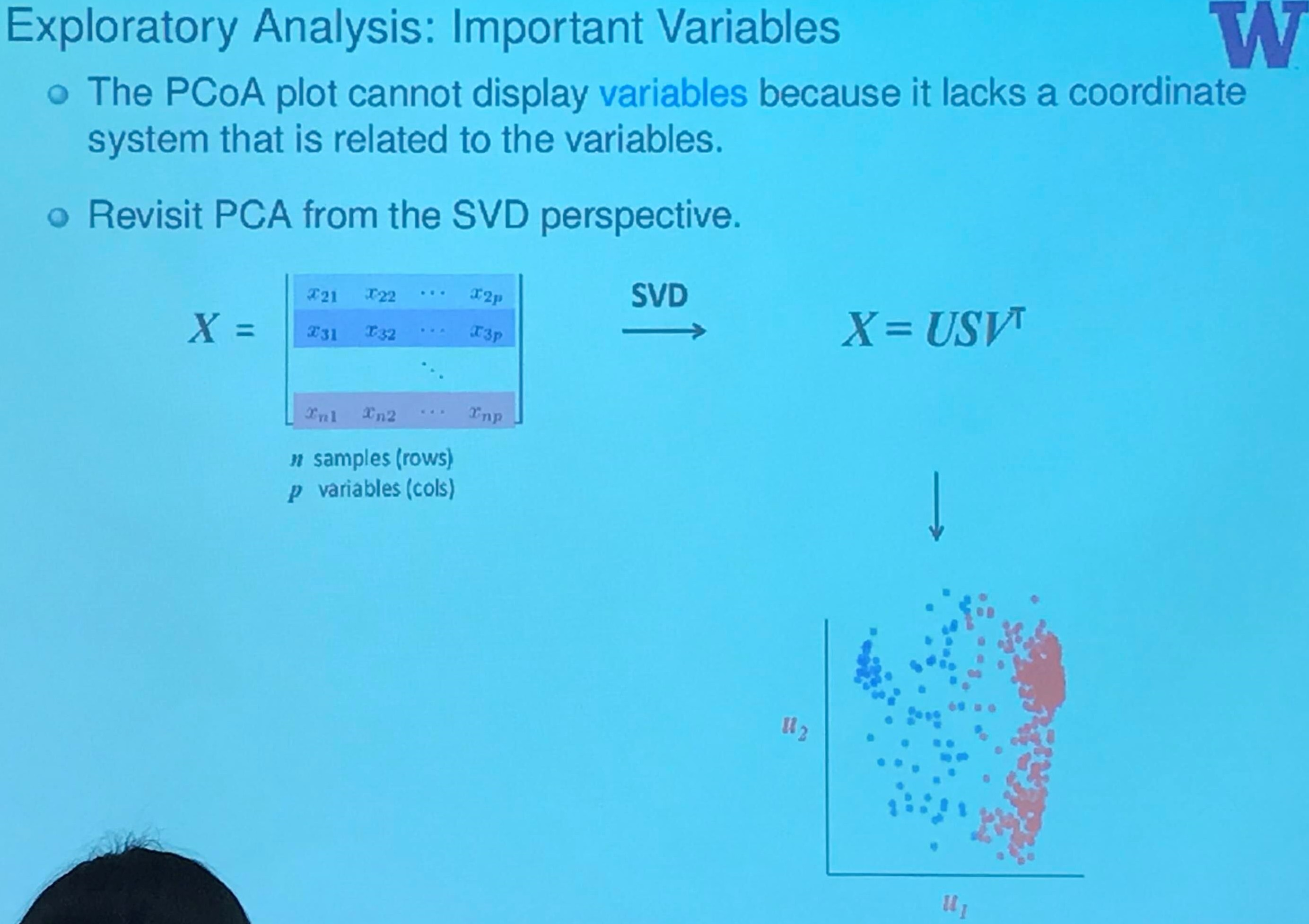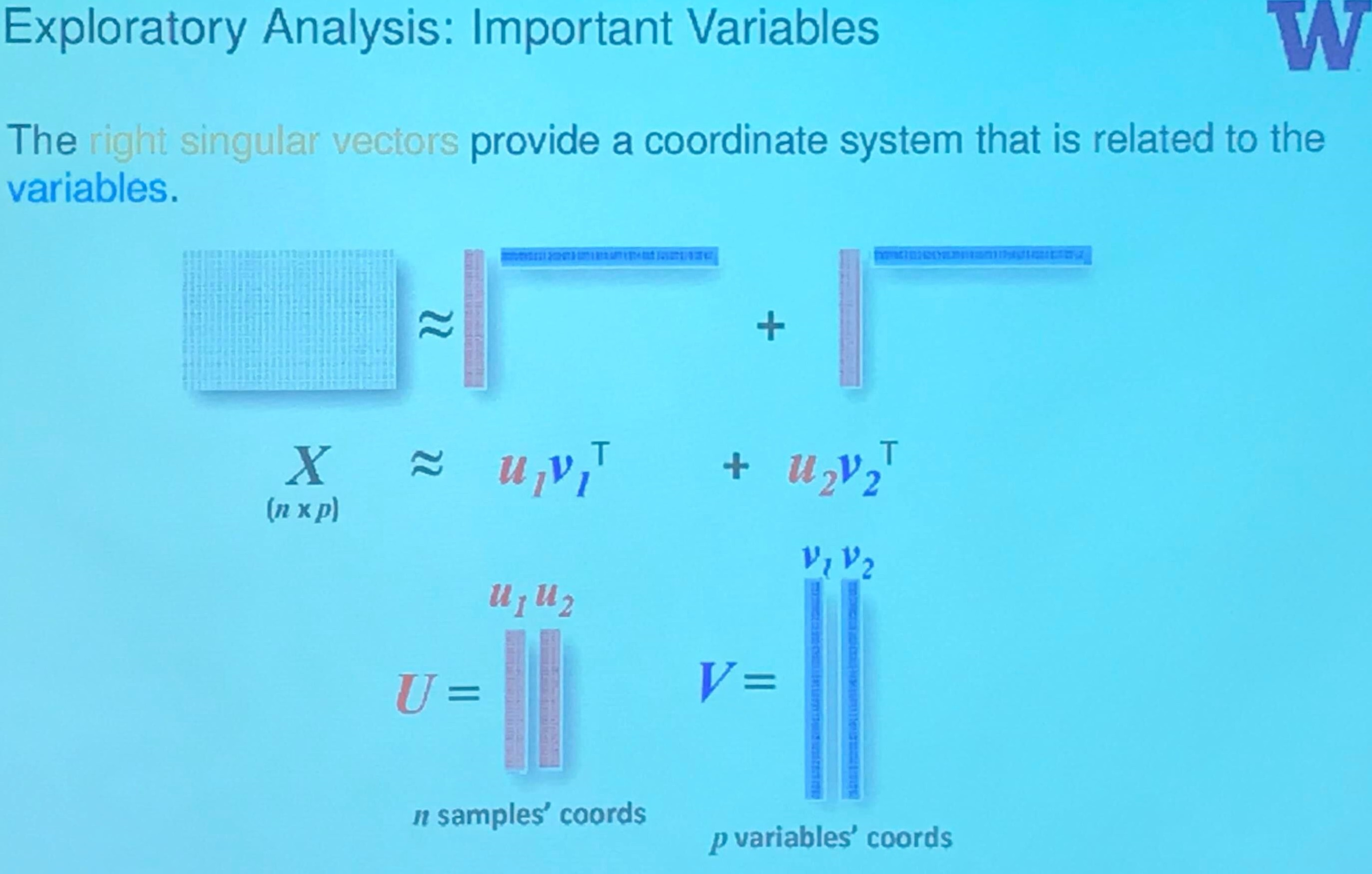If we consider them in separate coordinate system respectively, then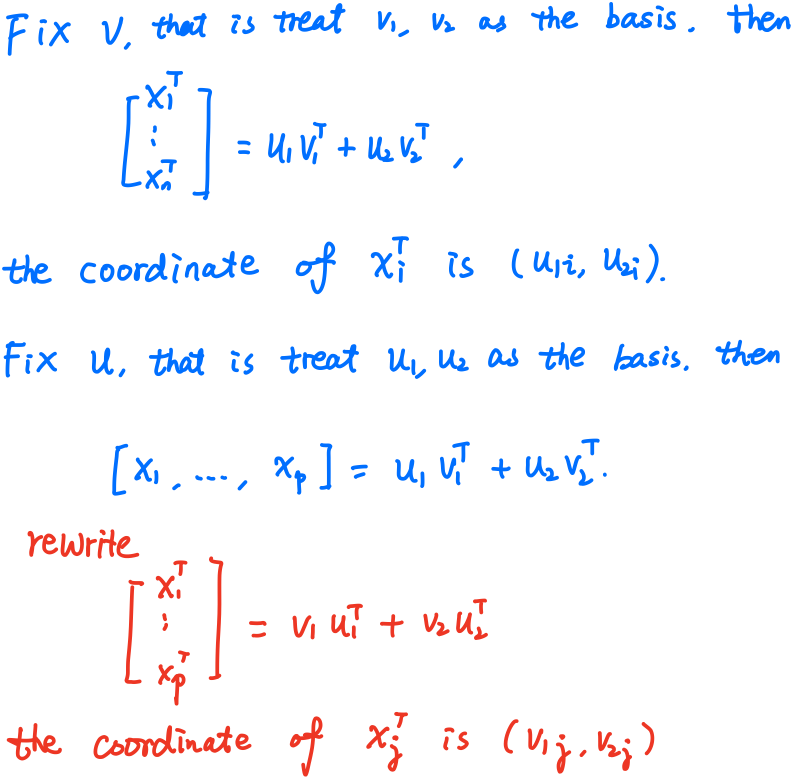but if we put them into a single coordinate system, then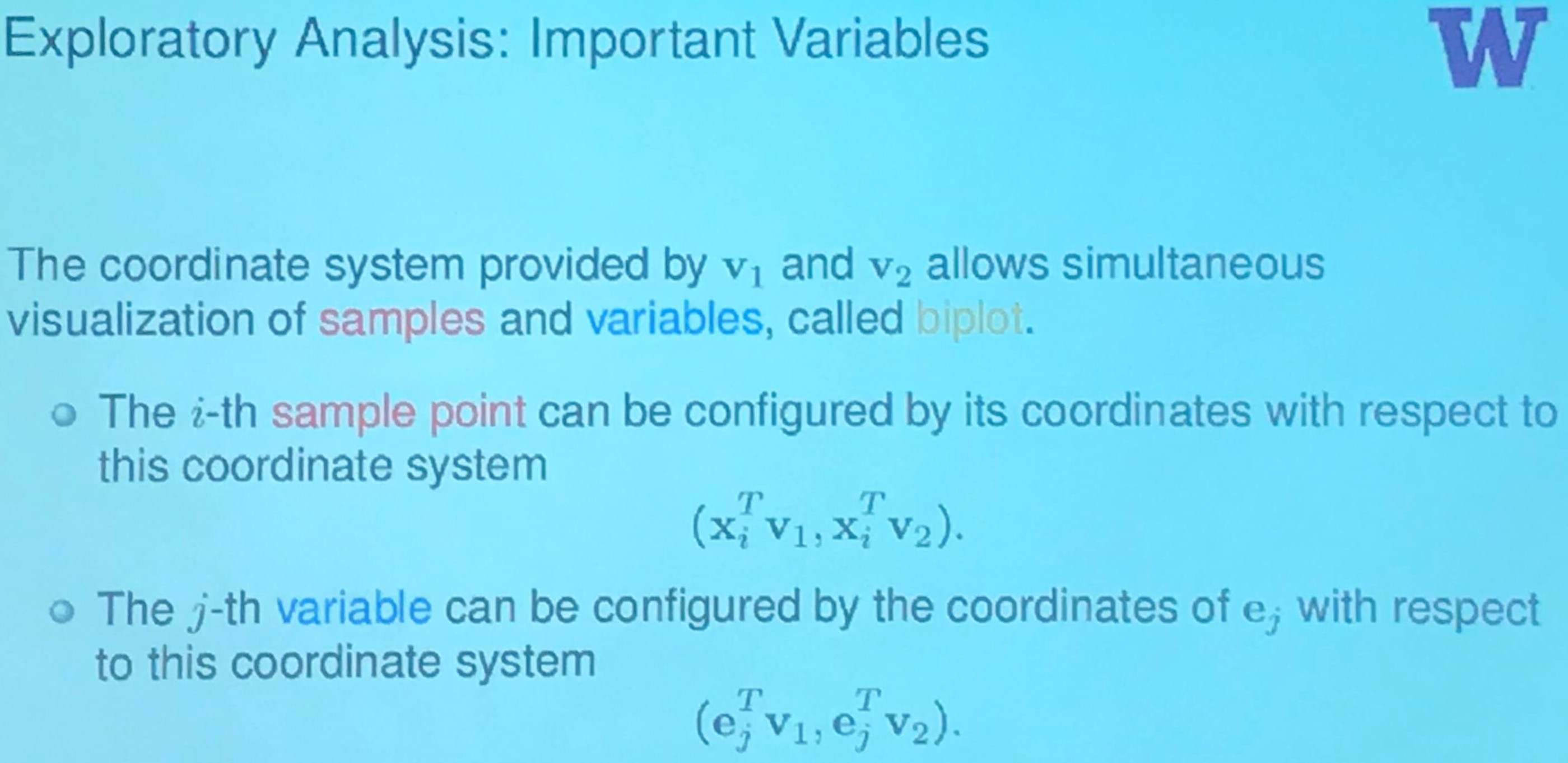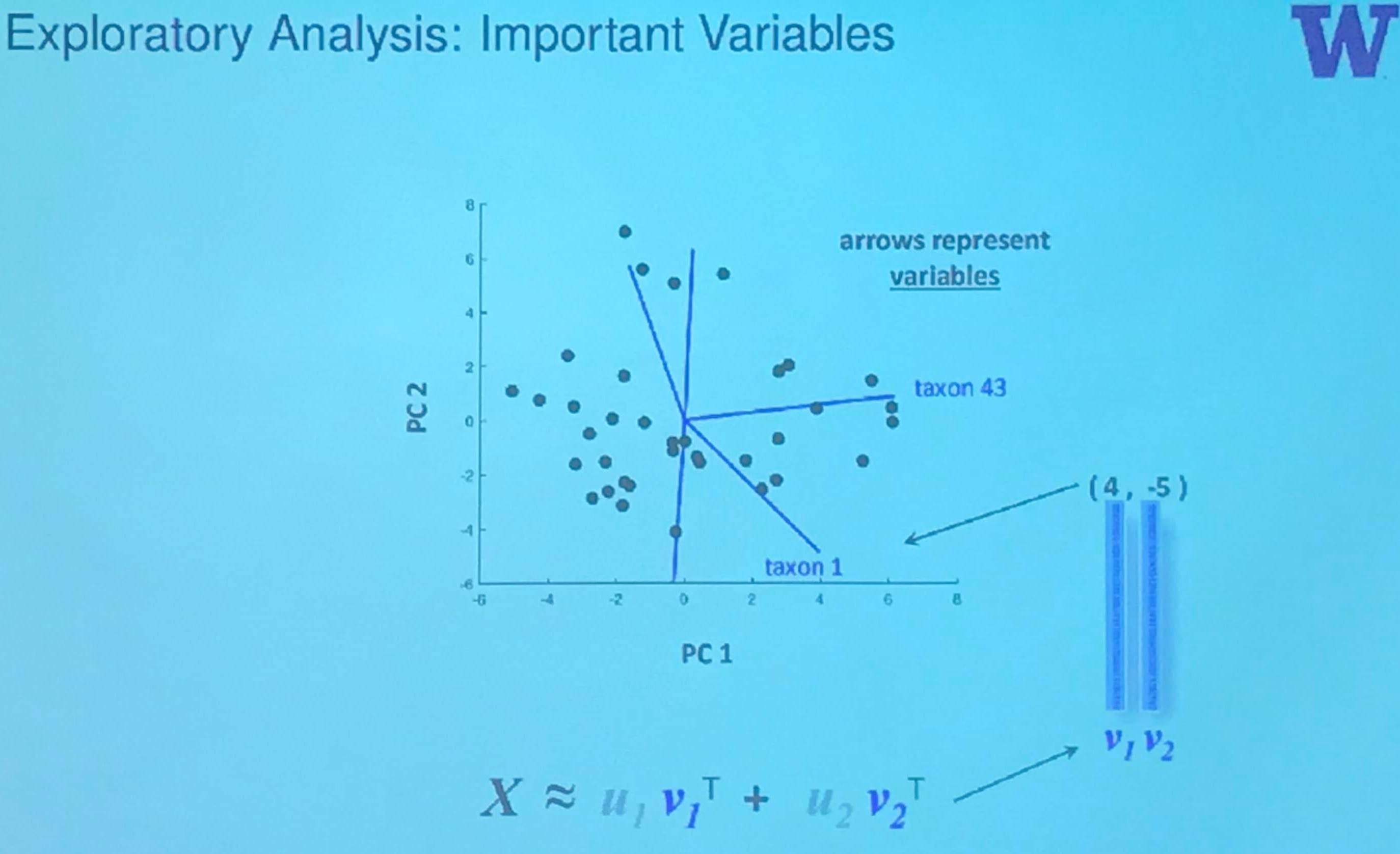where the biplot is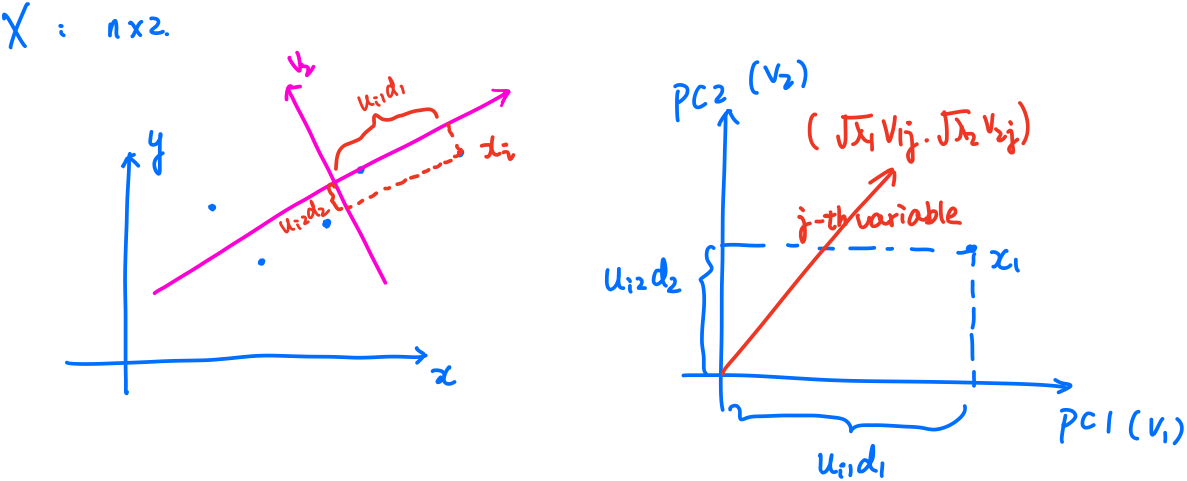## The GMD-biplot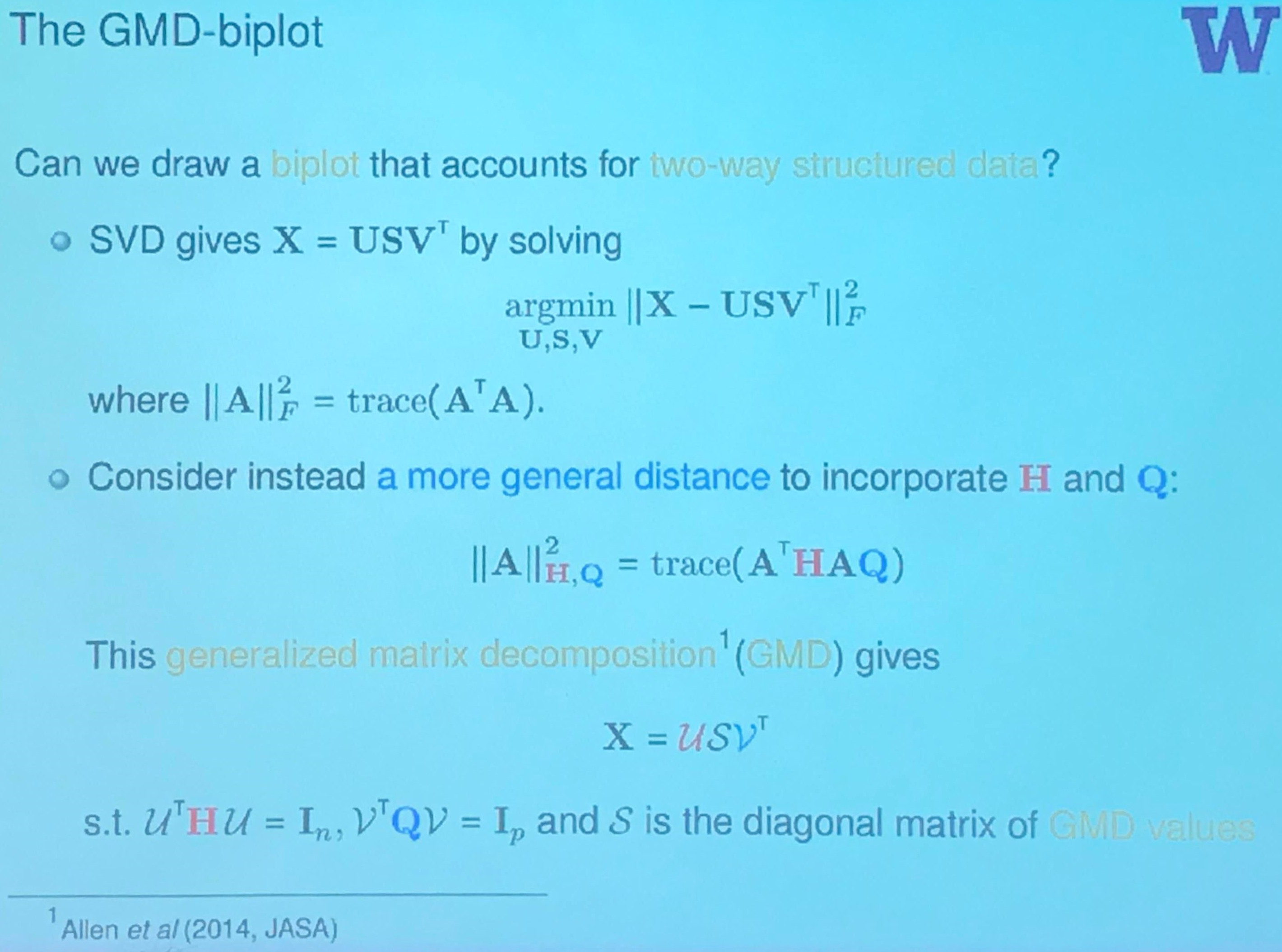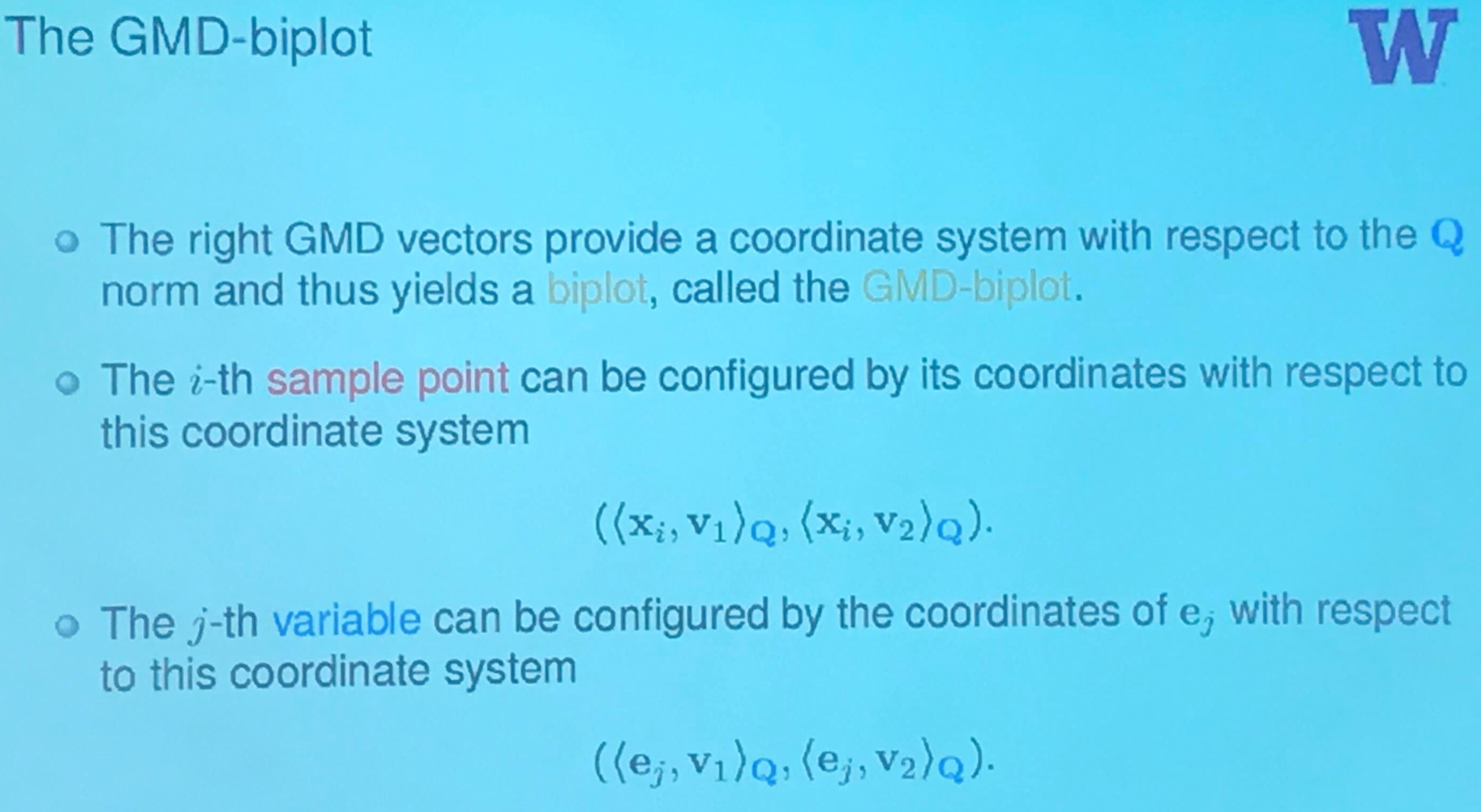## Smokeless Tobacco Data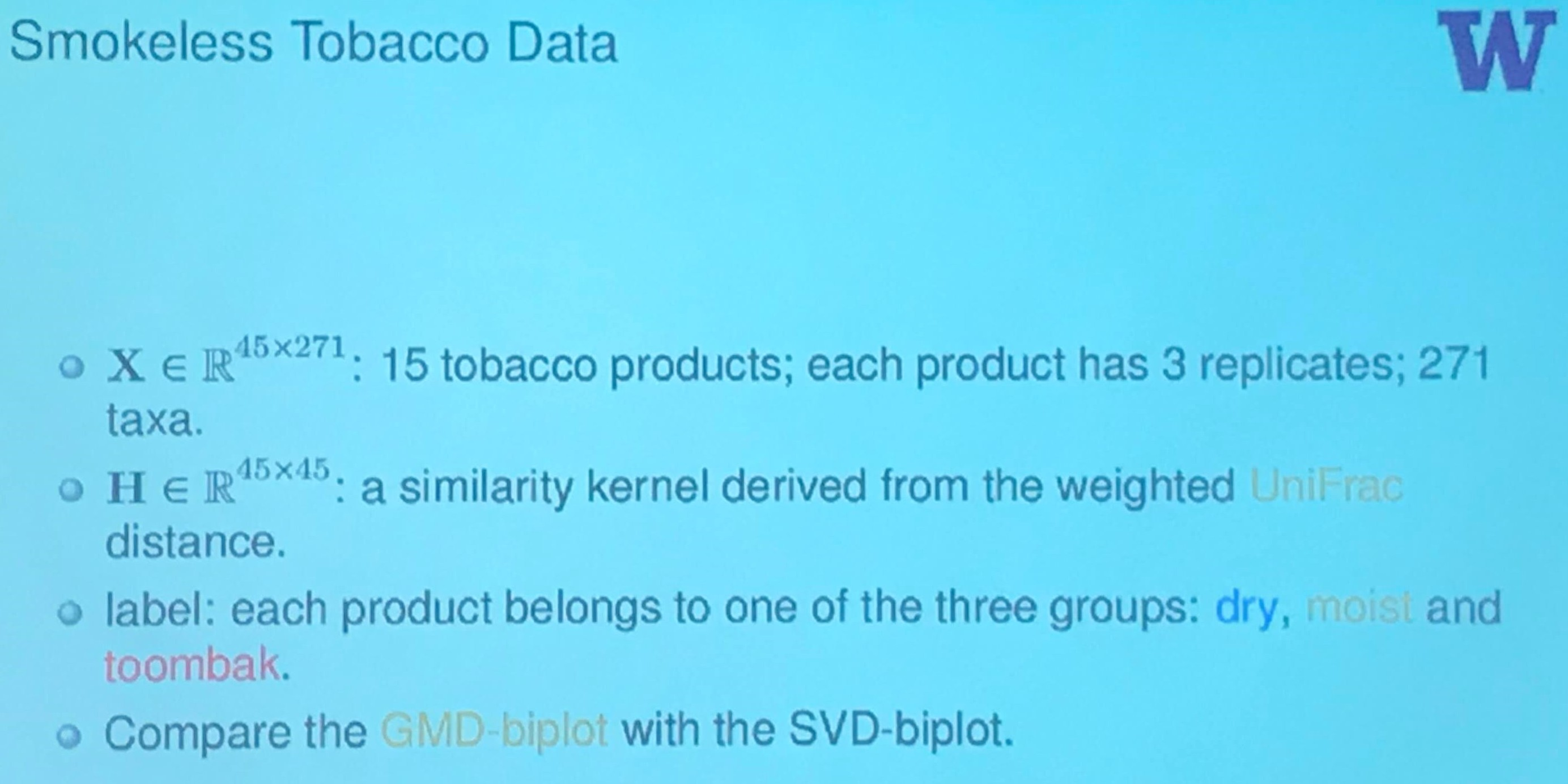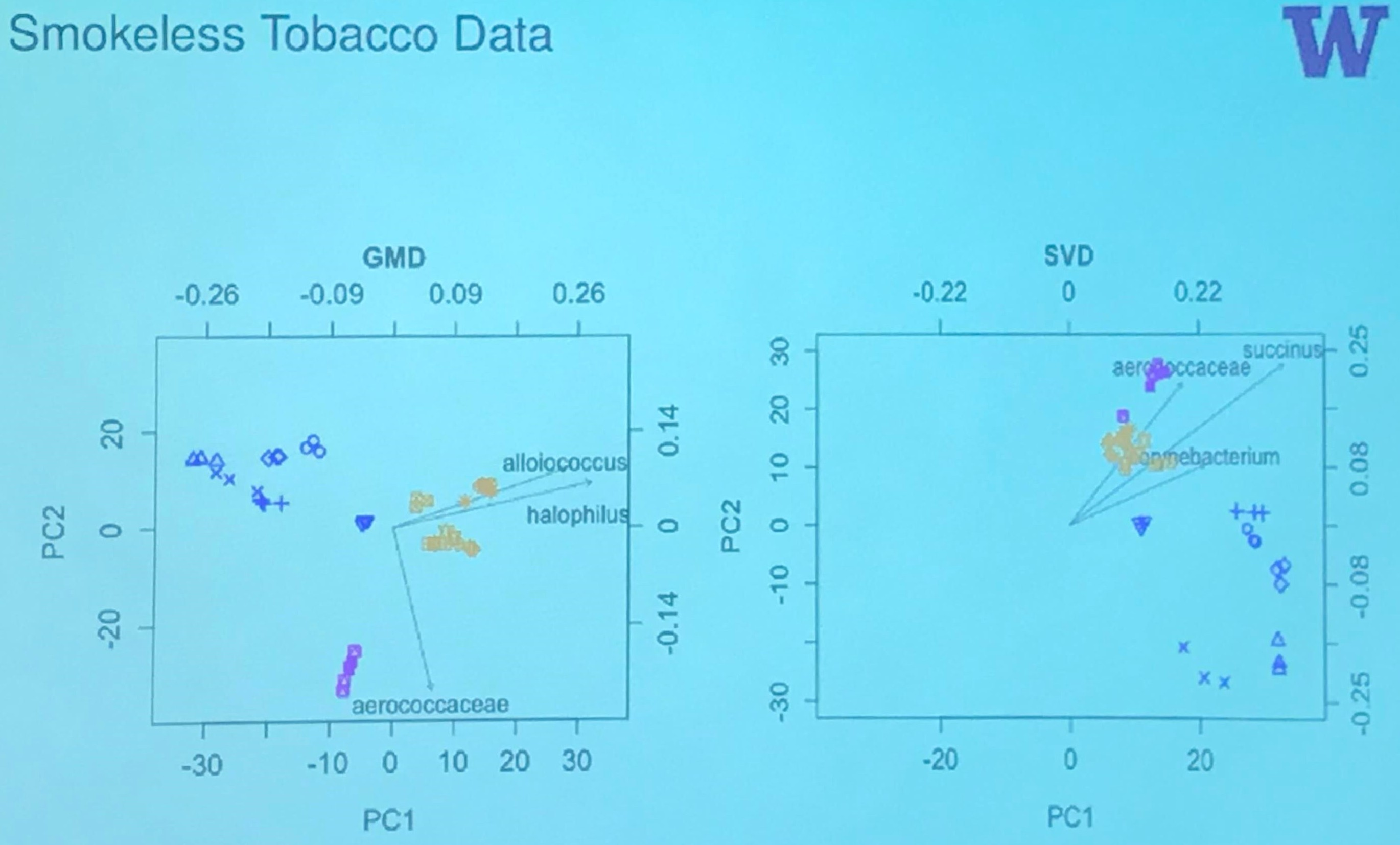The author also writes a tutorial for the GMD-biplot.

## Supervised Learning with the GMD: GMDR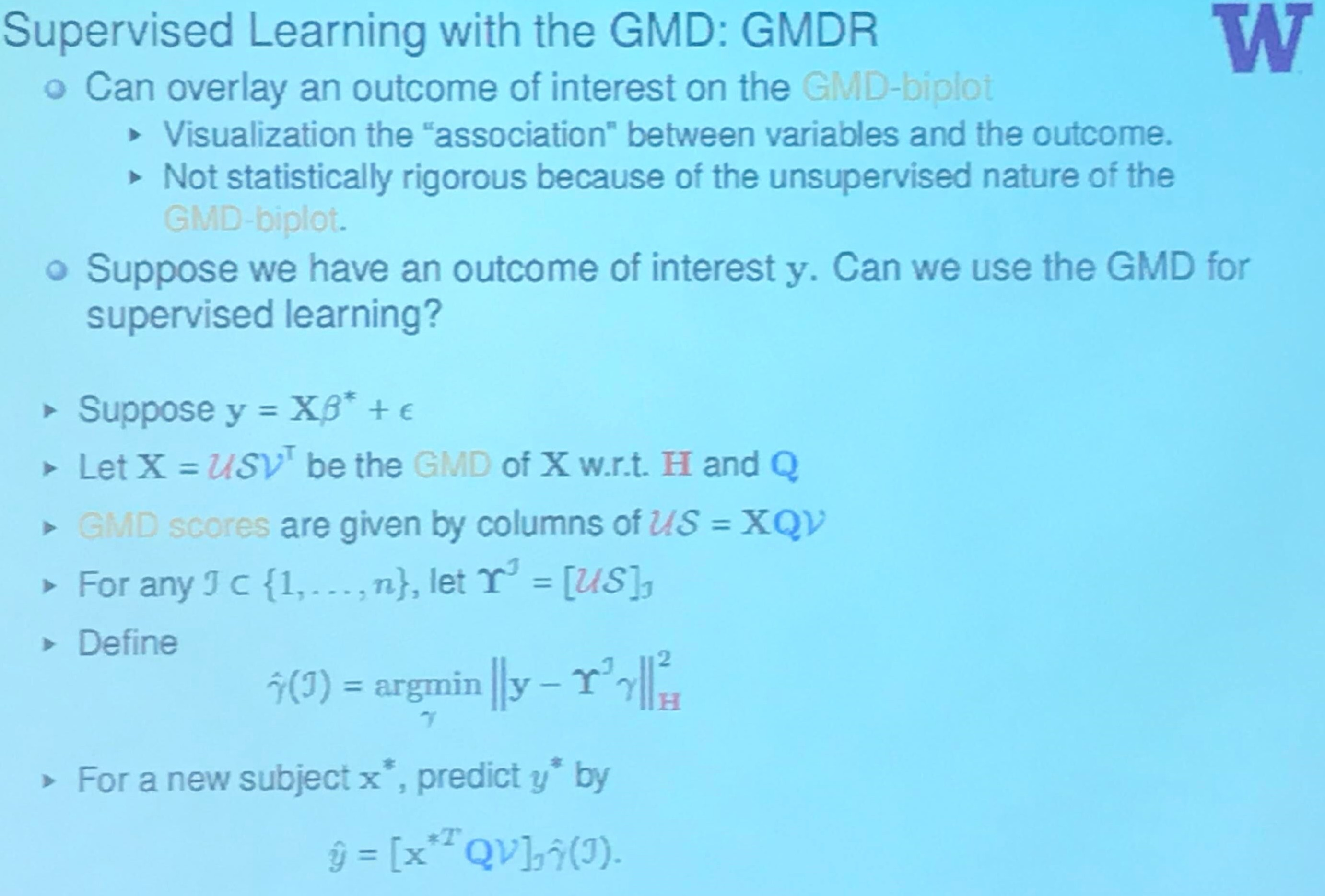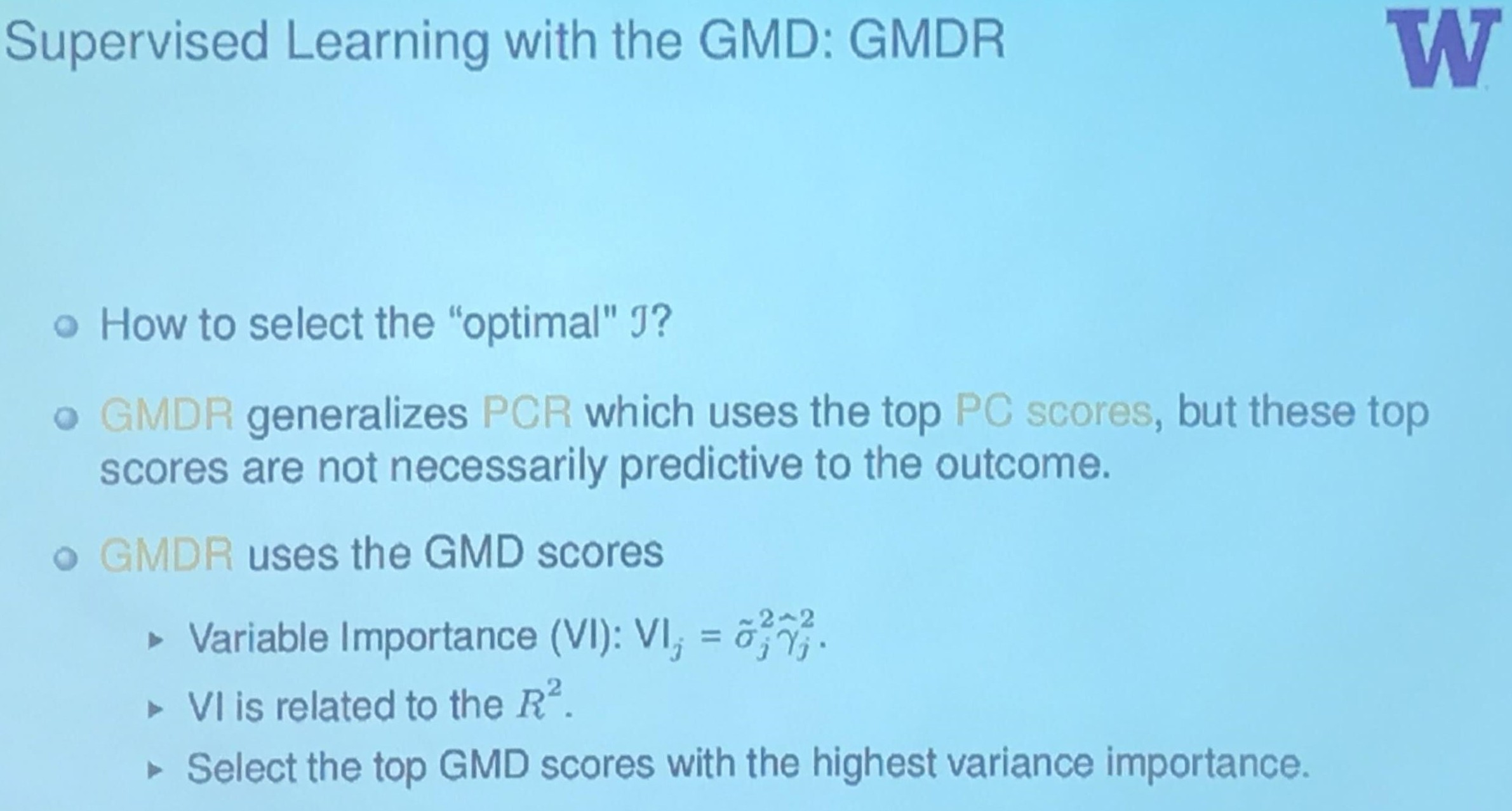where the variable importance is calculated using the response.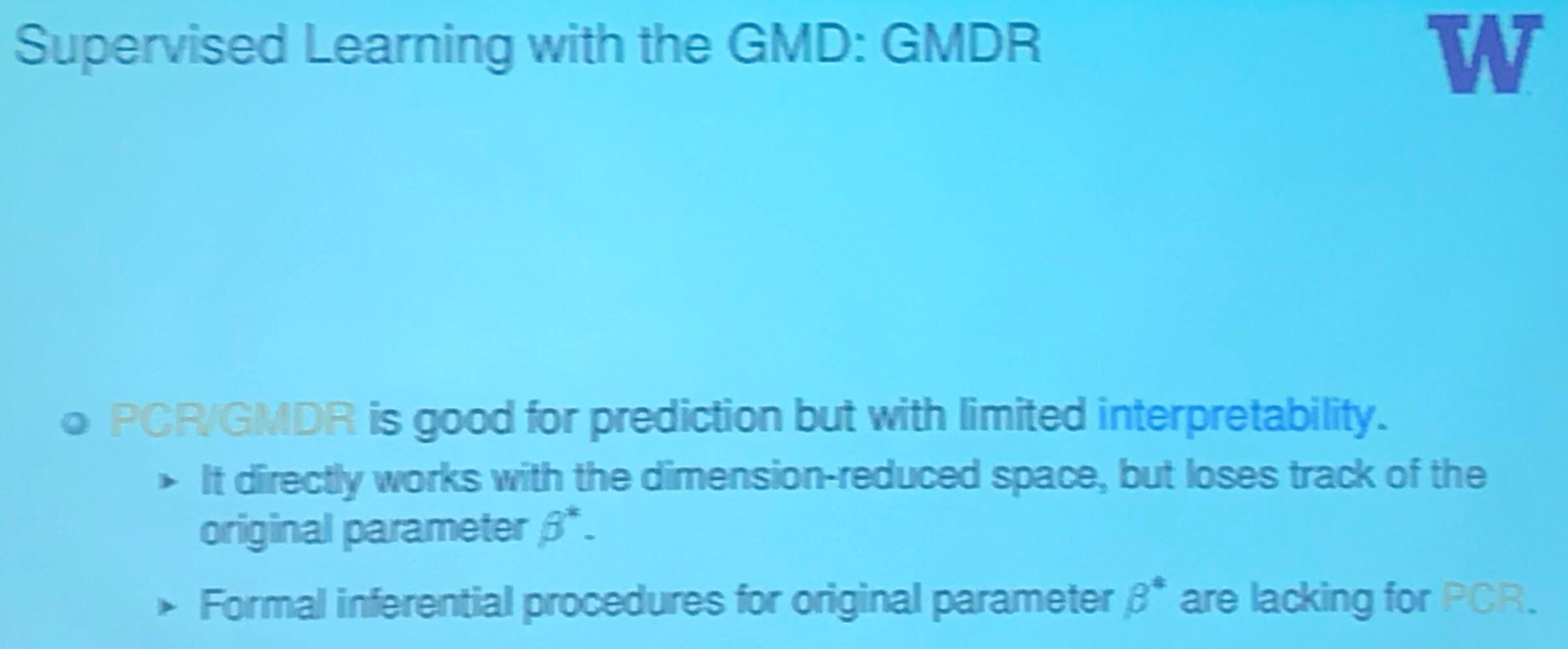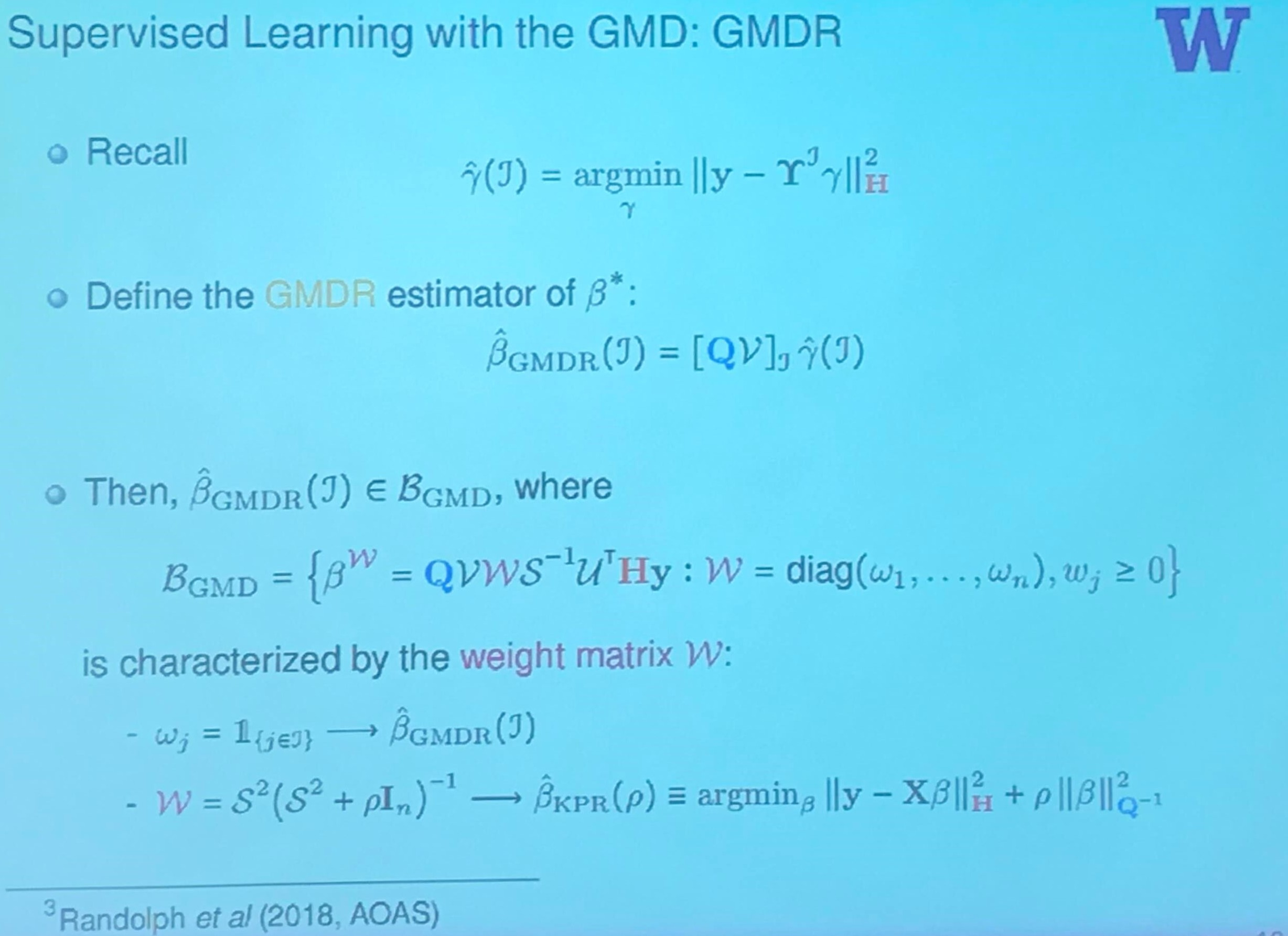why propose such a space of $\beta$? just want to exhibit the randomness of weights?

## Inference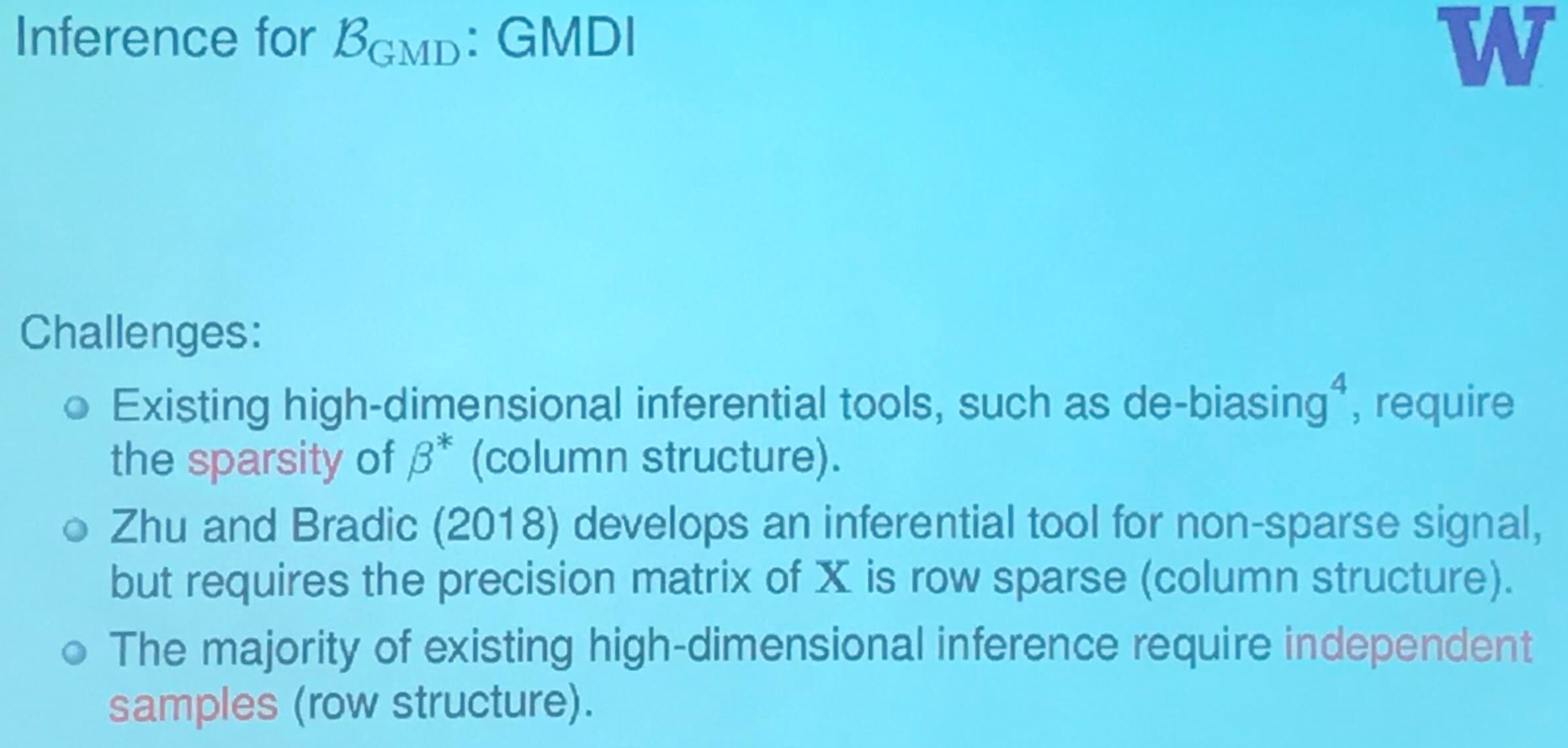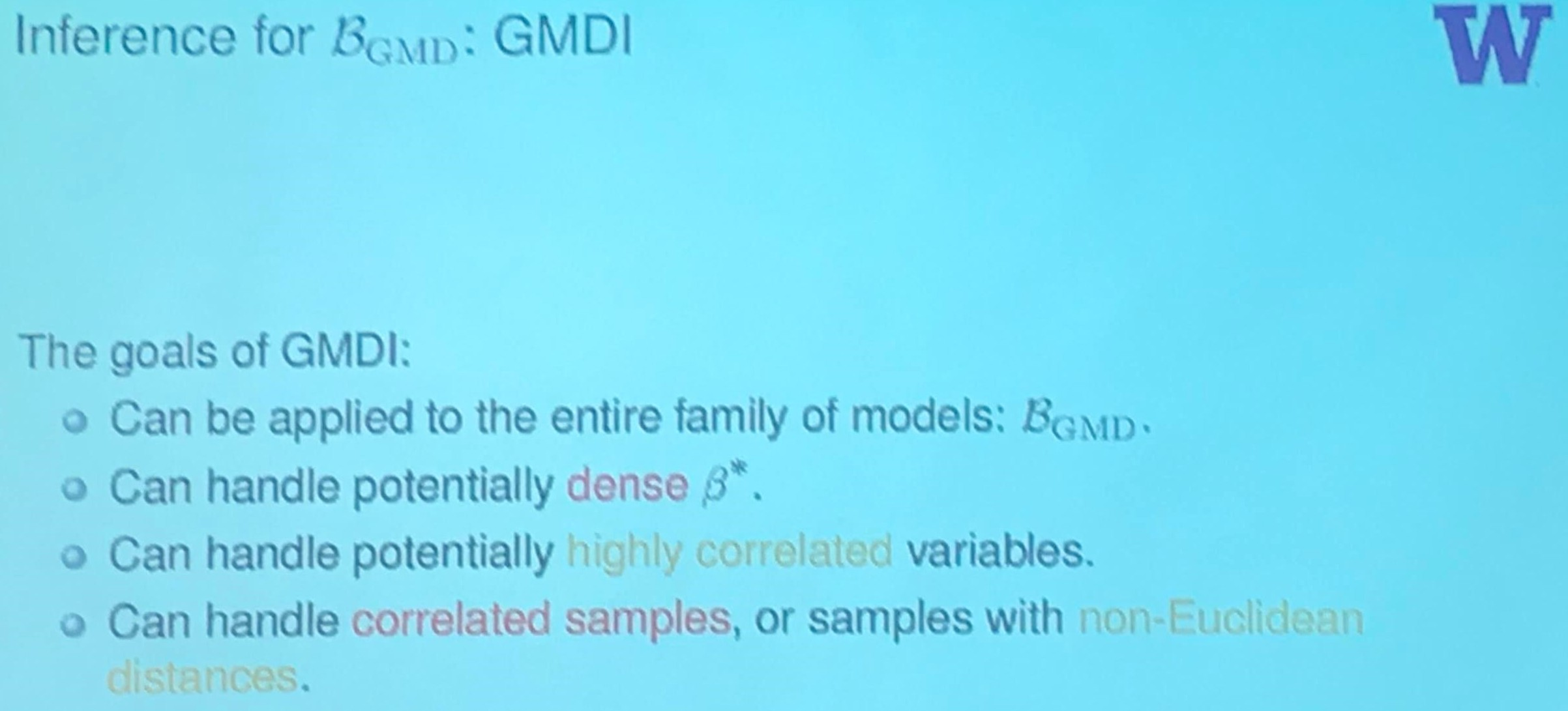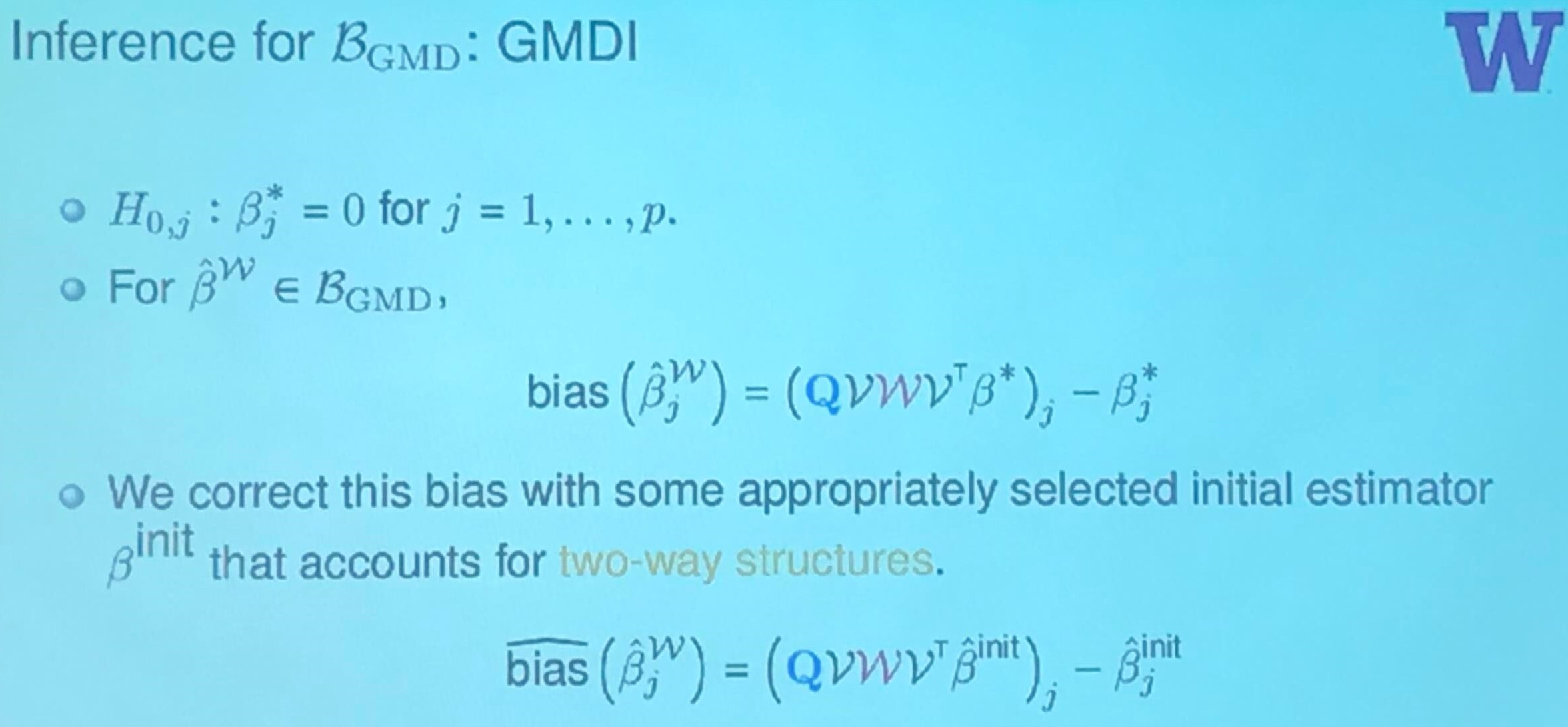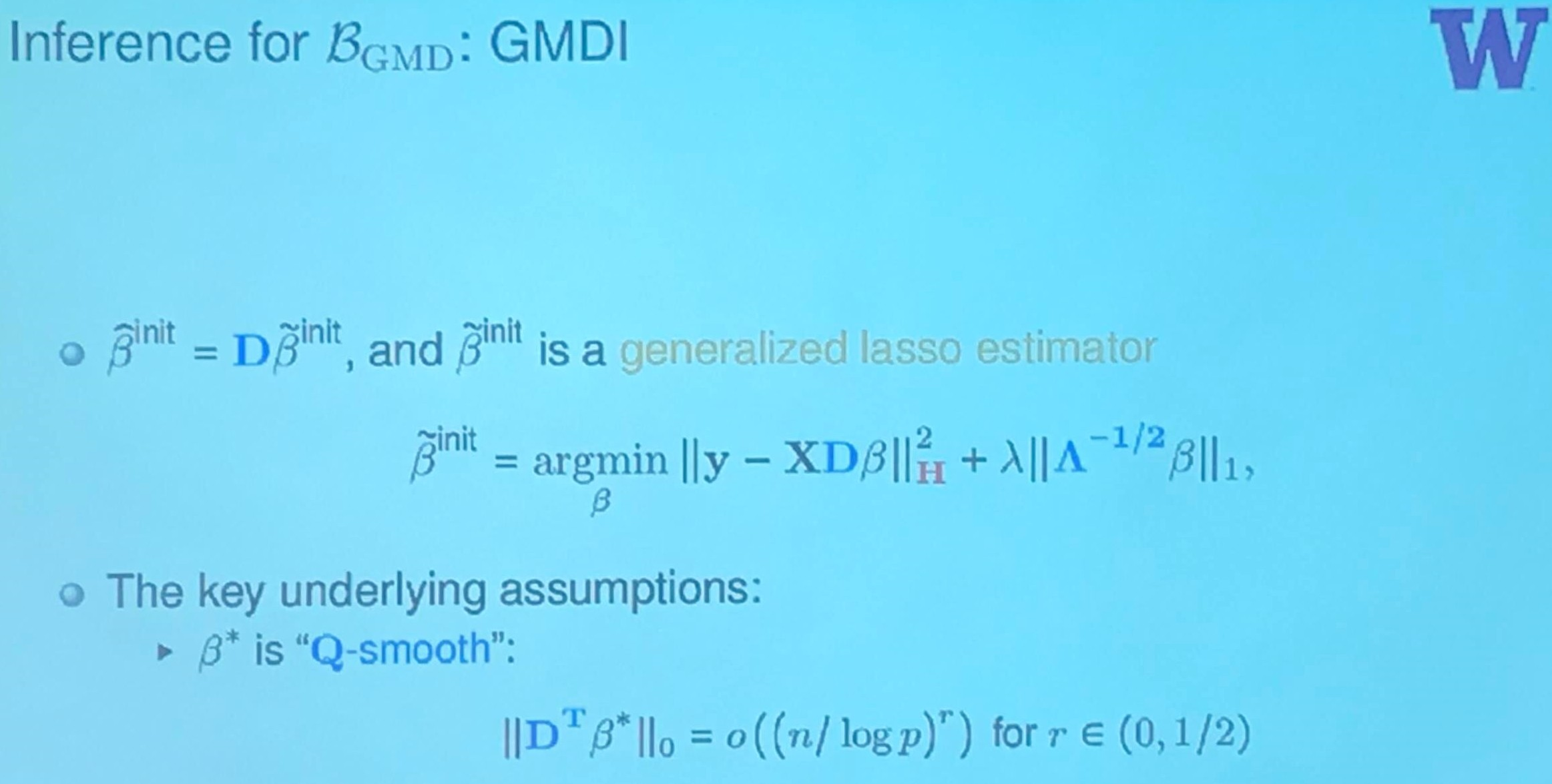what is $D$ here?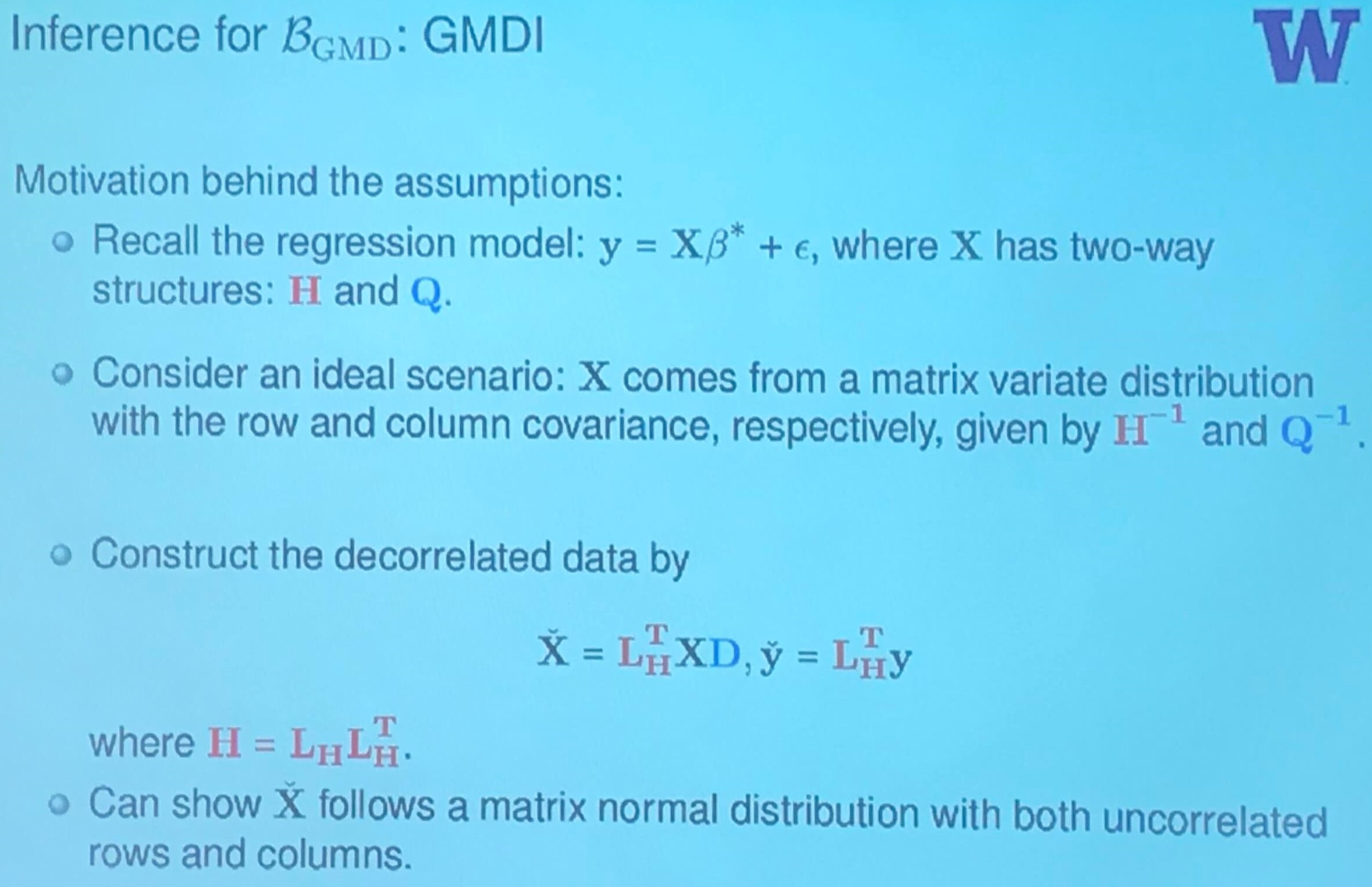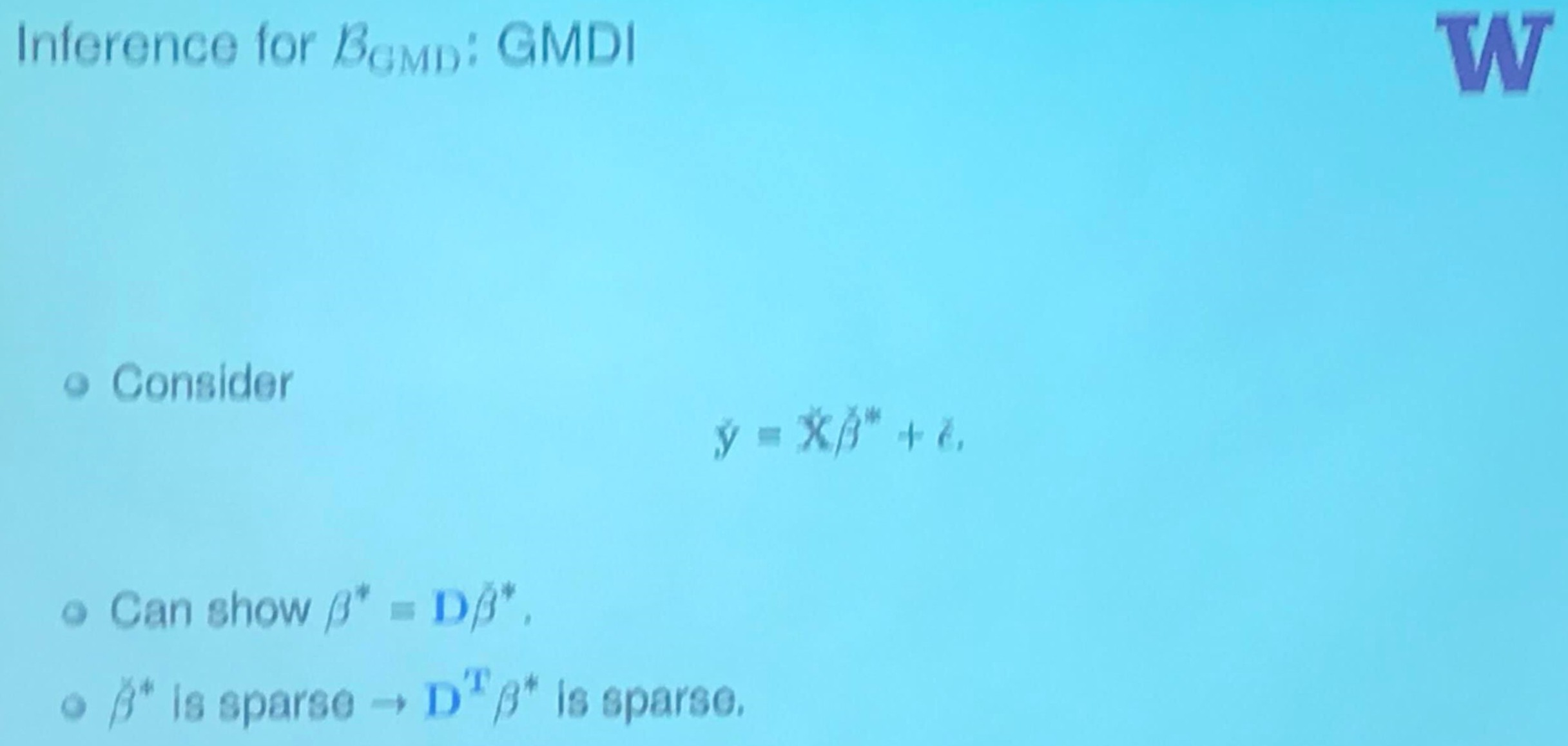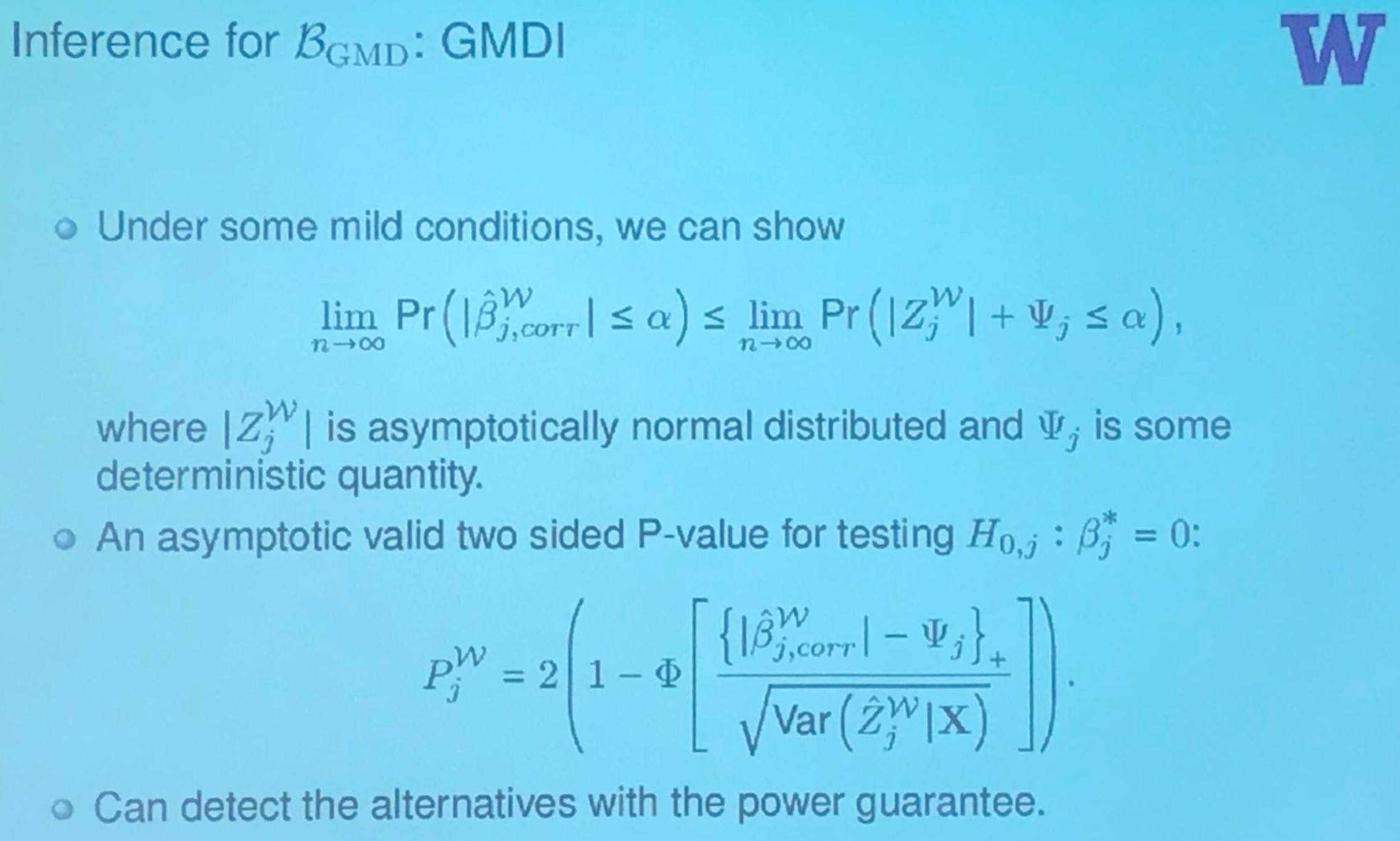## Human Gut Microbiome Data: Inference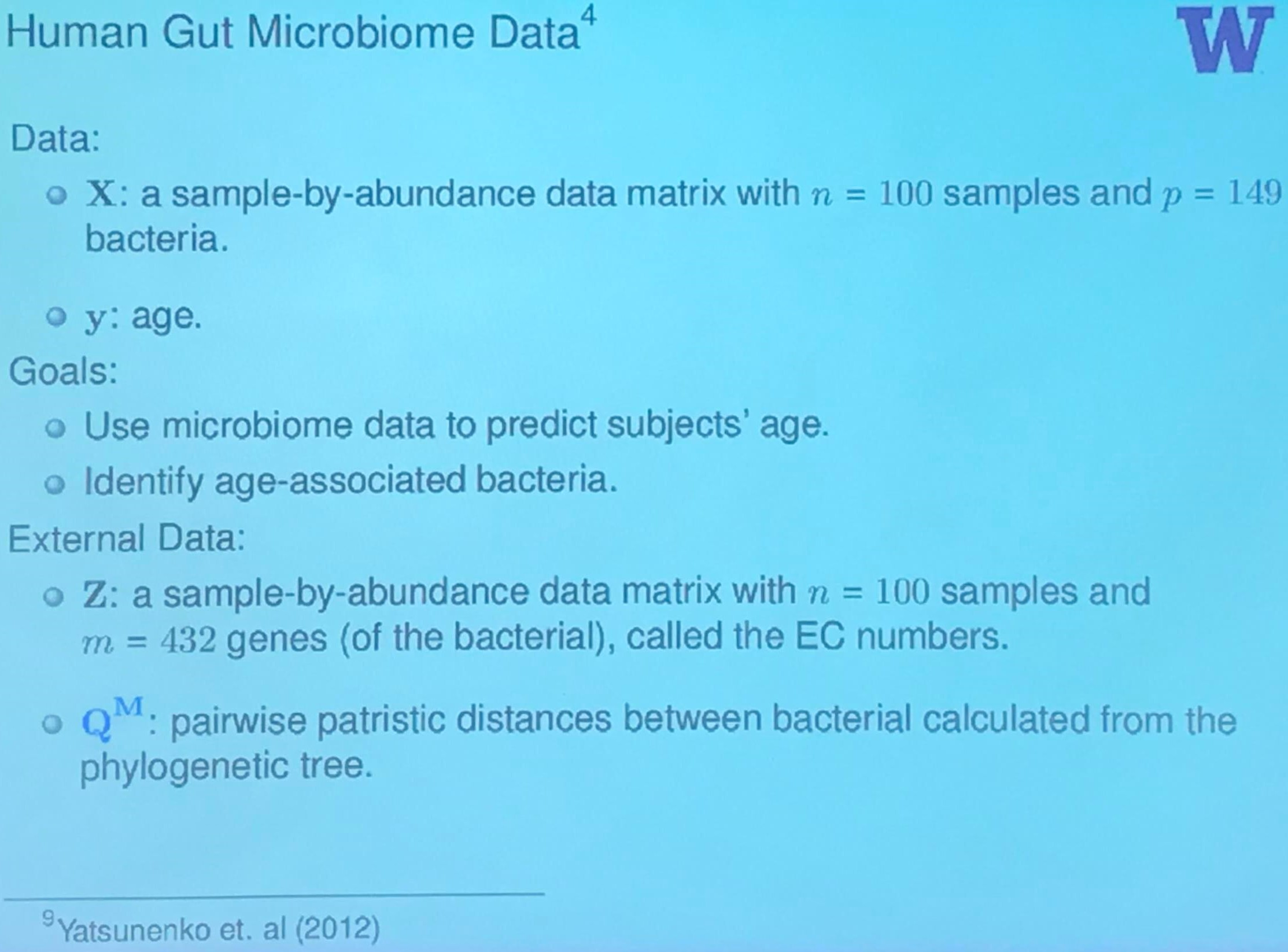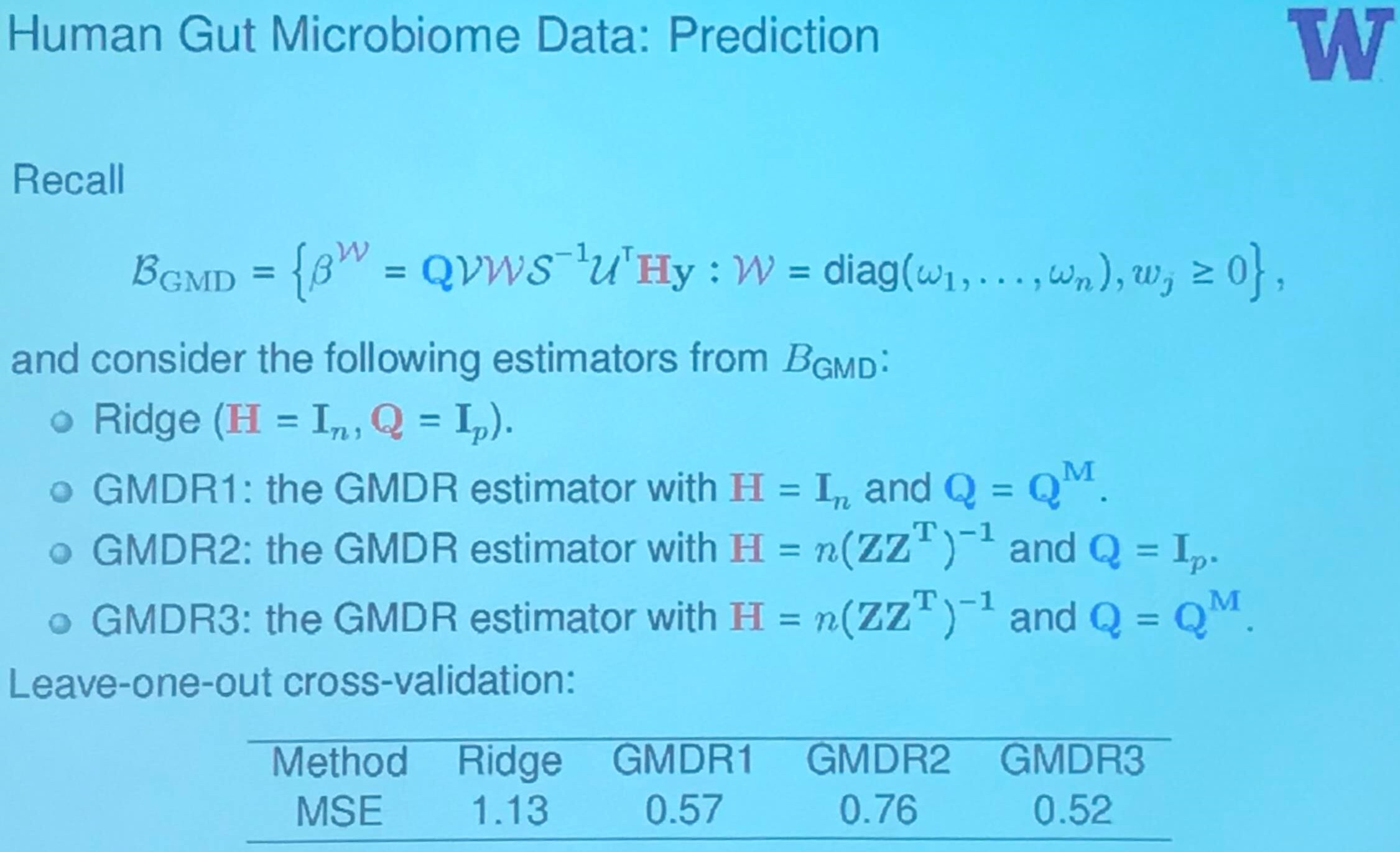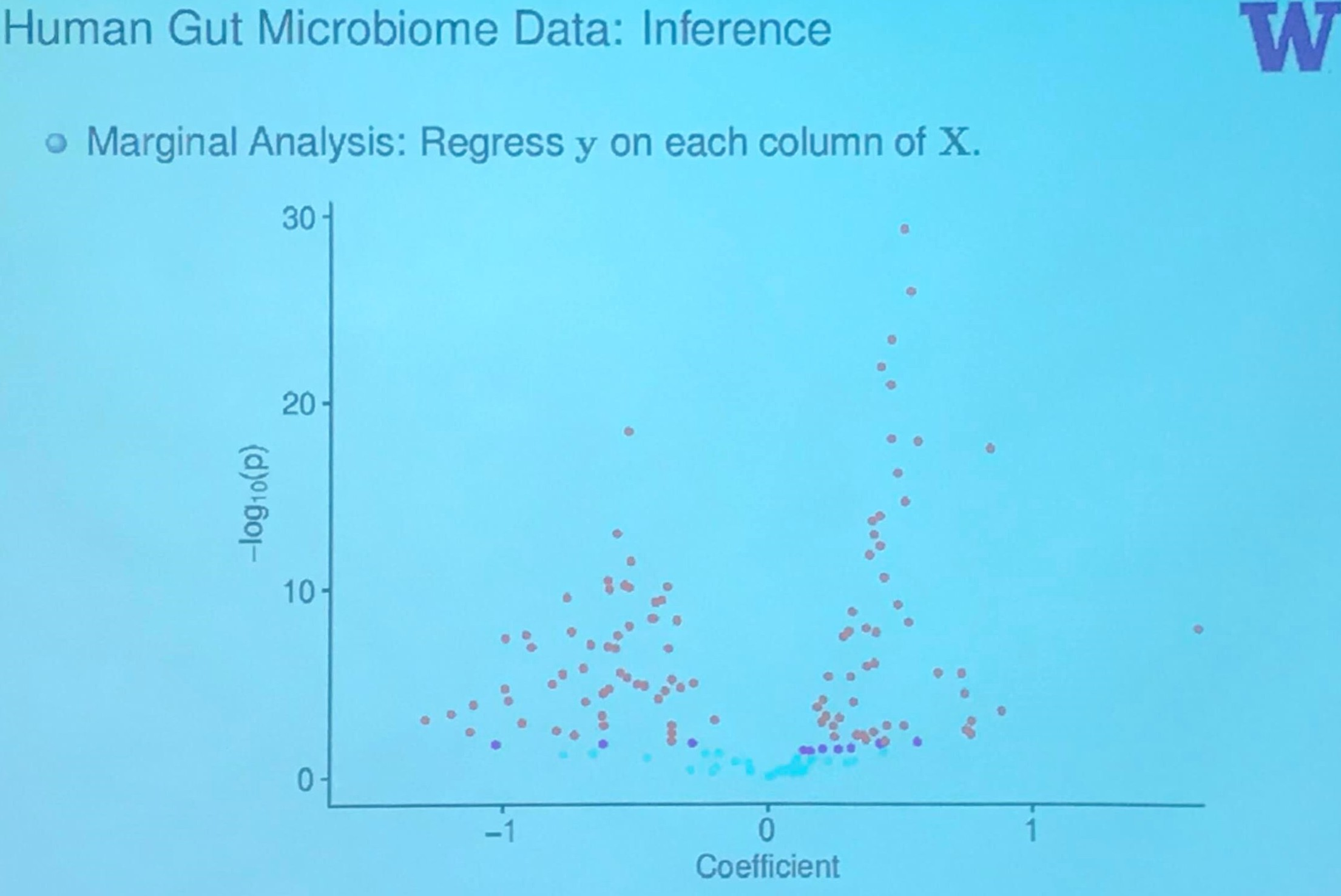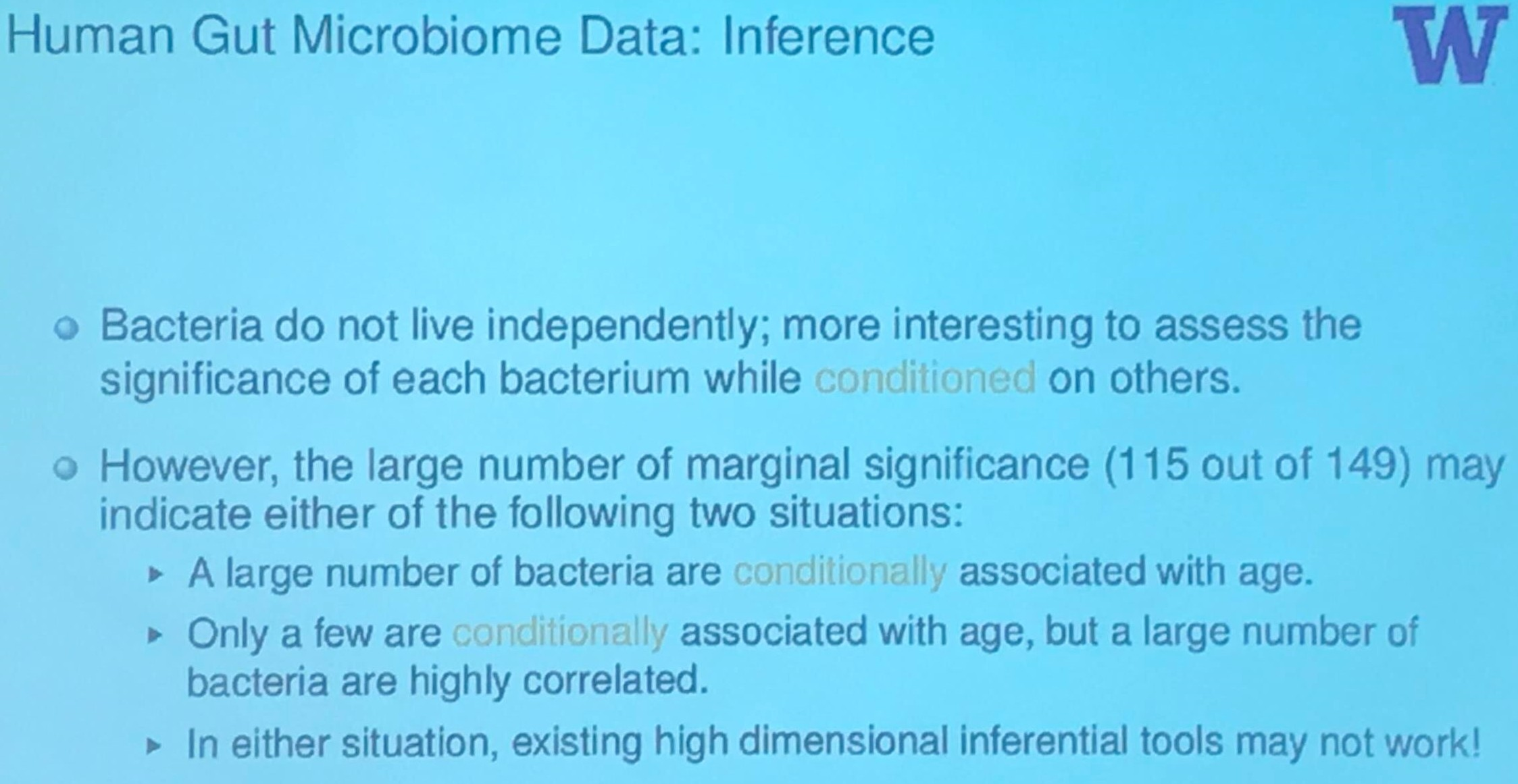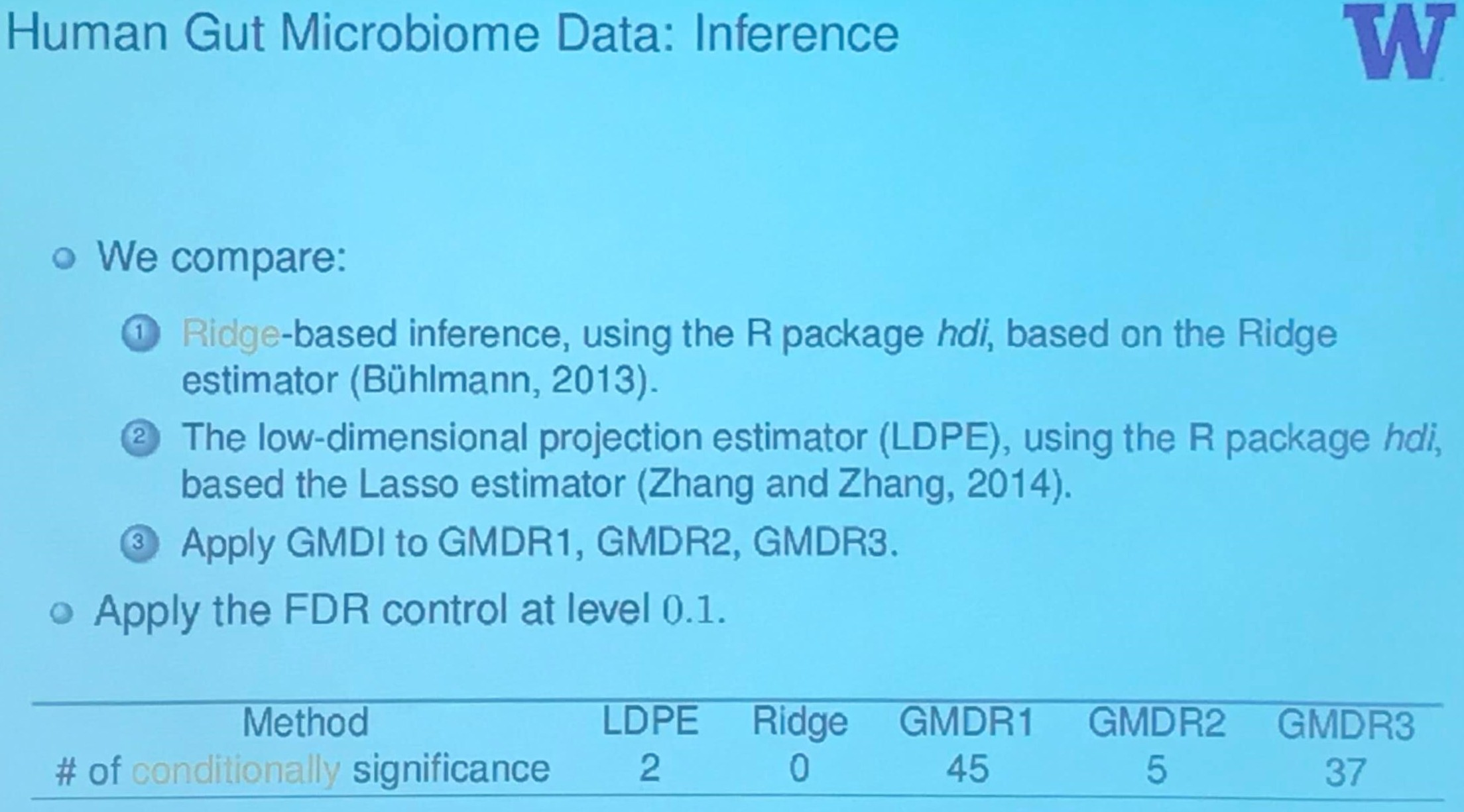## Summary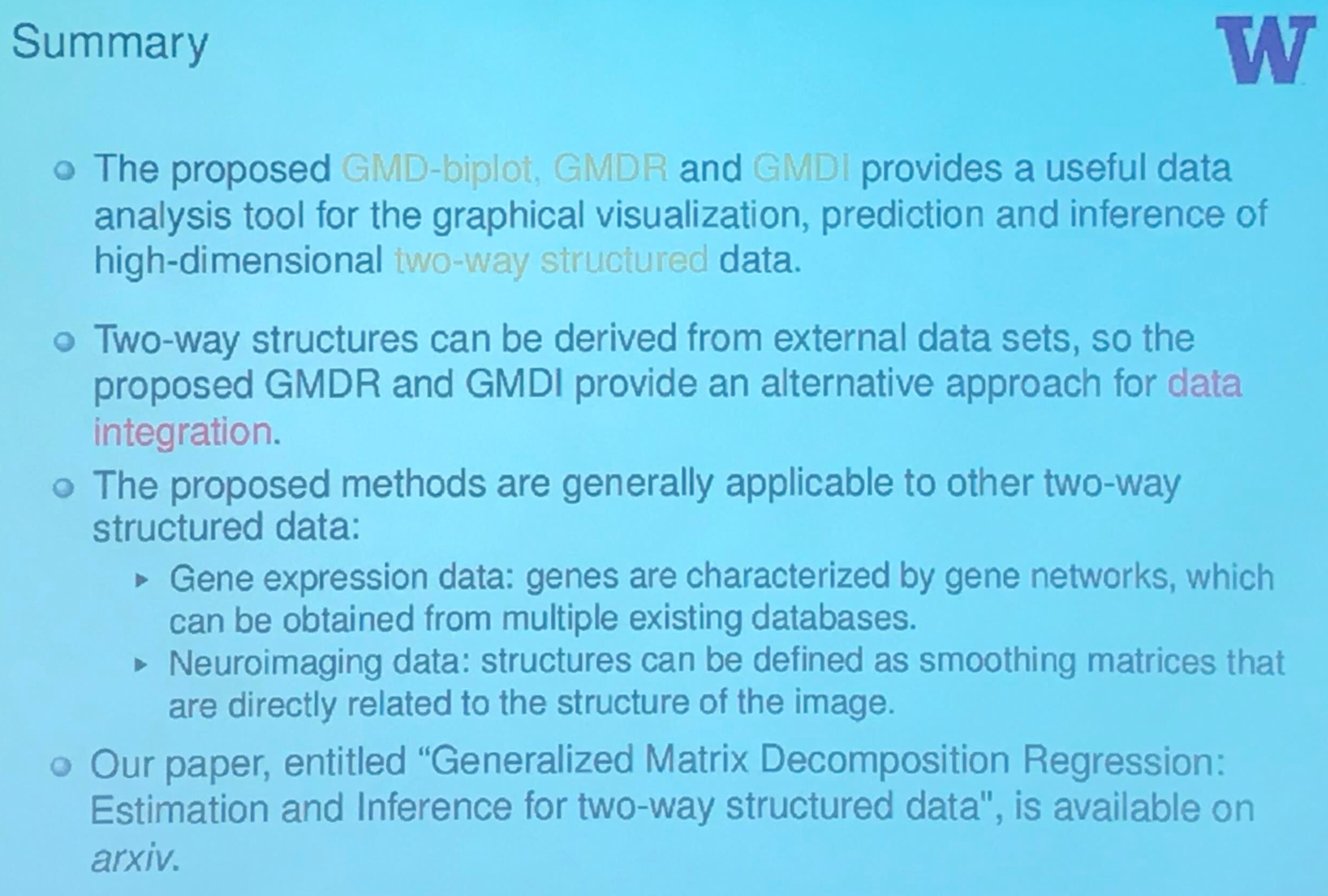Published in categories Seminar